# RS Aggarwal Solutions for Class 7 Maths Chapter 2 Fractions

RS Aggarwal Solutions for Class 7 Maths Chapter 2 – Fractions are provided here. To cover all the syllabus in Maths, the RS Aggarwal is an essential material as it offers a wide range of questions that test the students understanding of concepts. Our expert personnel have solved the problems step by step with neat explanations. Students who want to score good marks in Maths, then refer RS Aggarwal Class 7 Solutions.

Expert tutors at BYJU’S have prepared the RS Aggarwal Solutions for Class 7 Maths Chapter 2, and it contains all exercises. Students gain more knowledge by referring to RS Aggarwal Solutions. Download pdf of Class 7 Chapter 2 in their respective links.

## Download PDF of RS Aggarwal Solutions for Class 7 Maths Chapter 2 – Fractions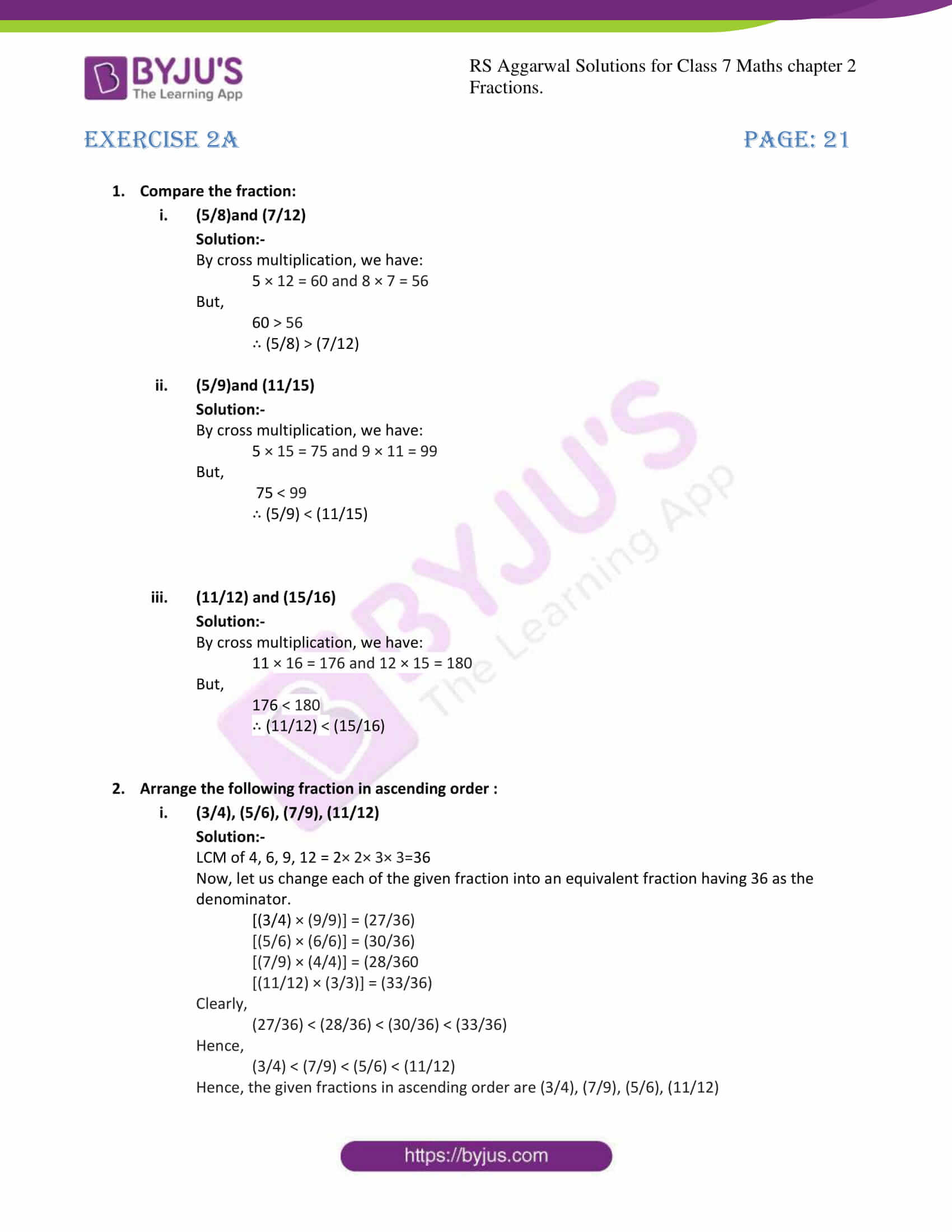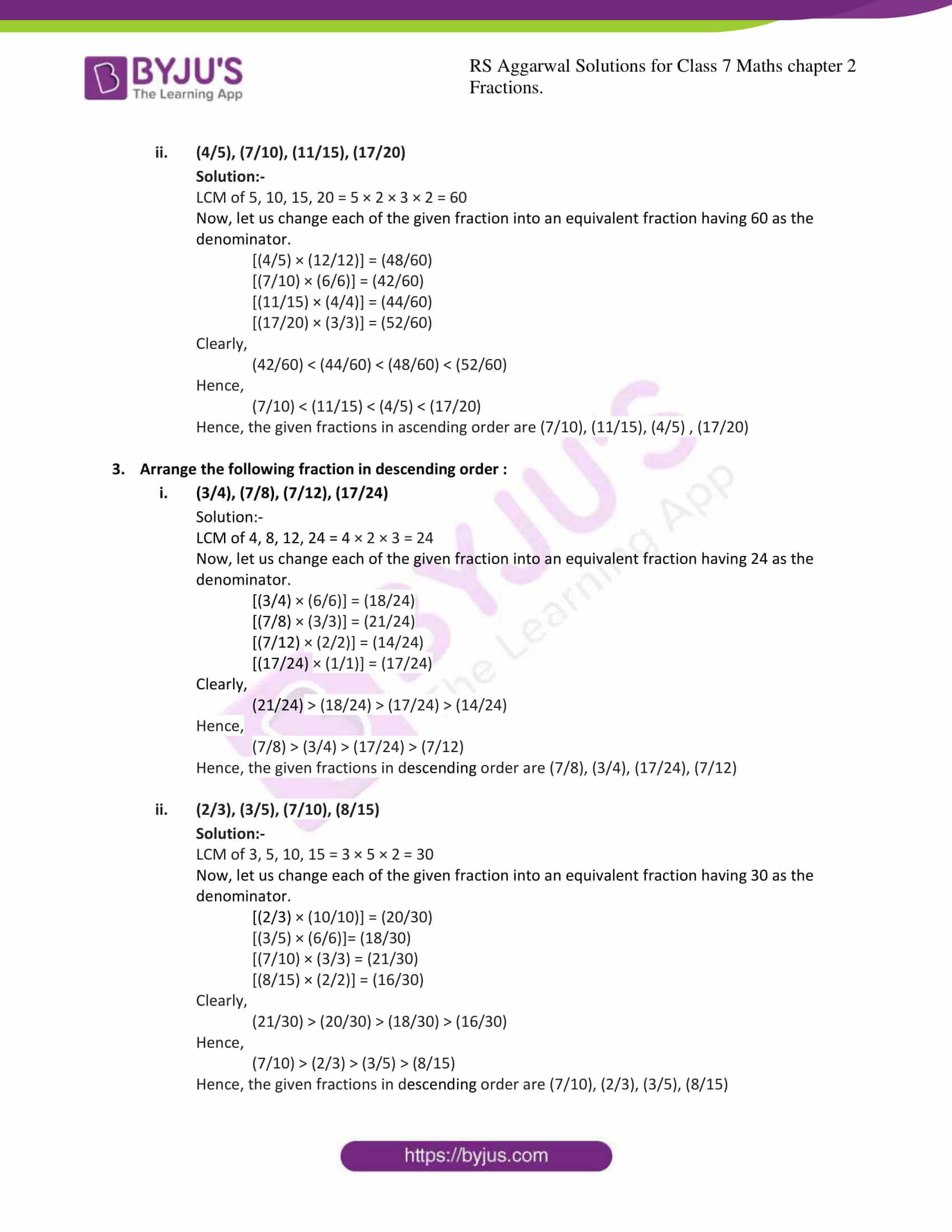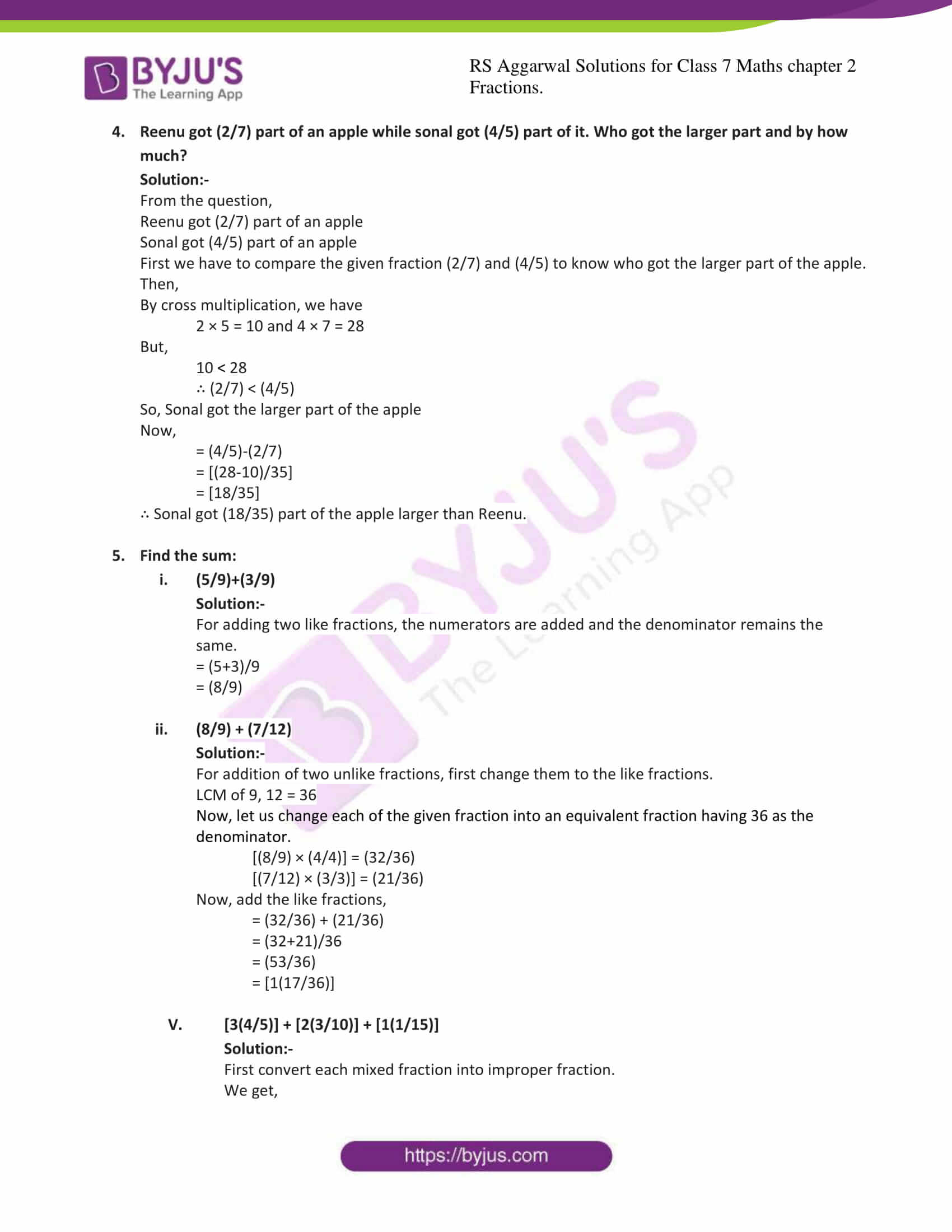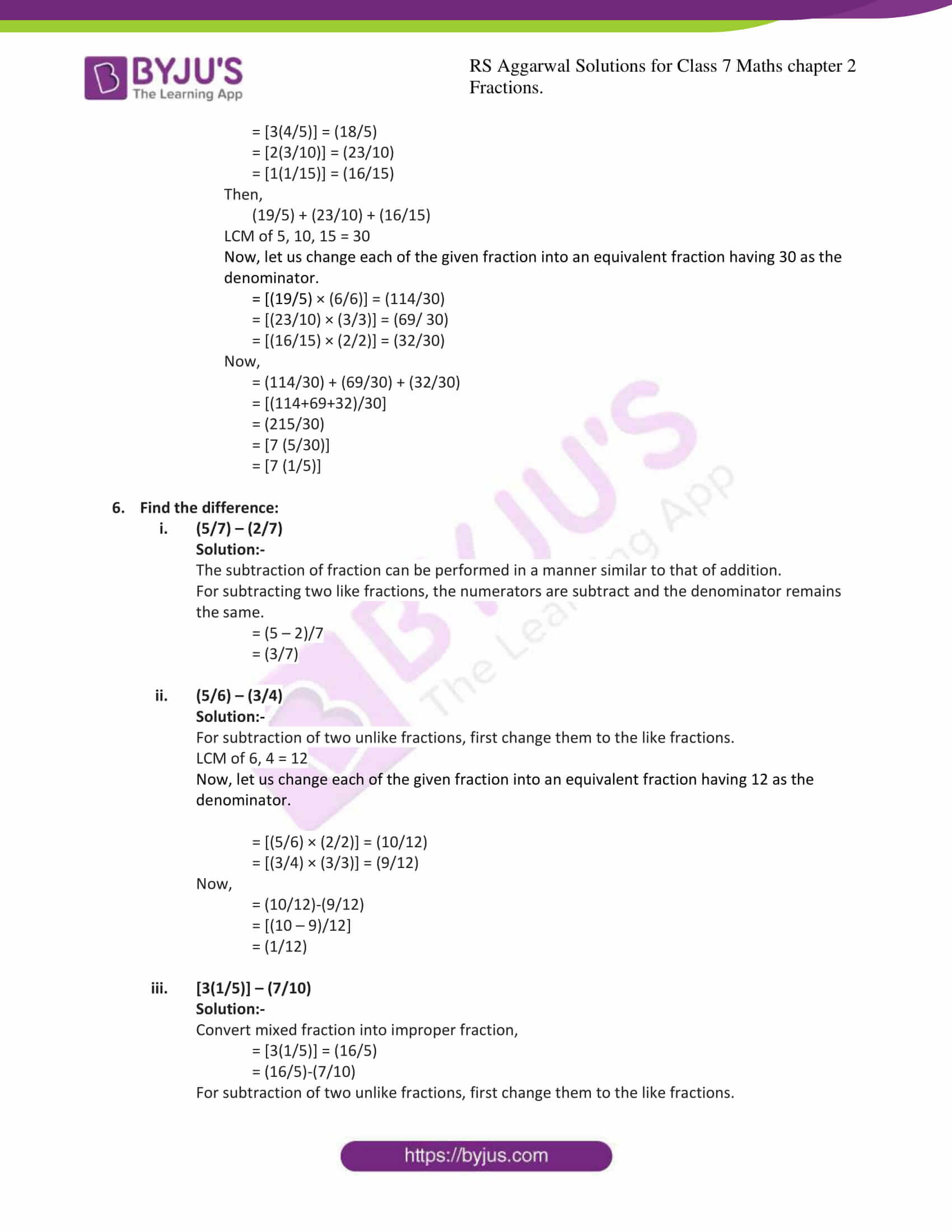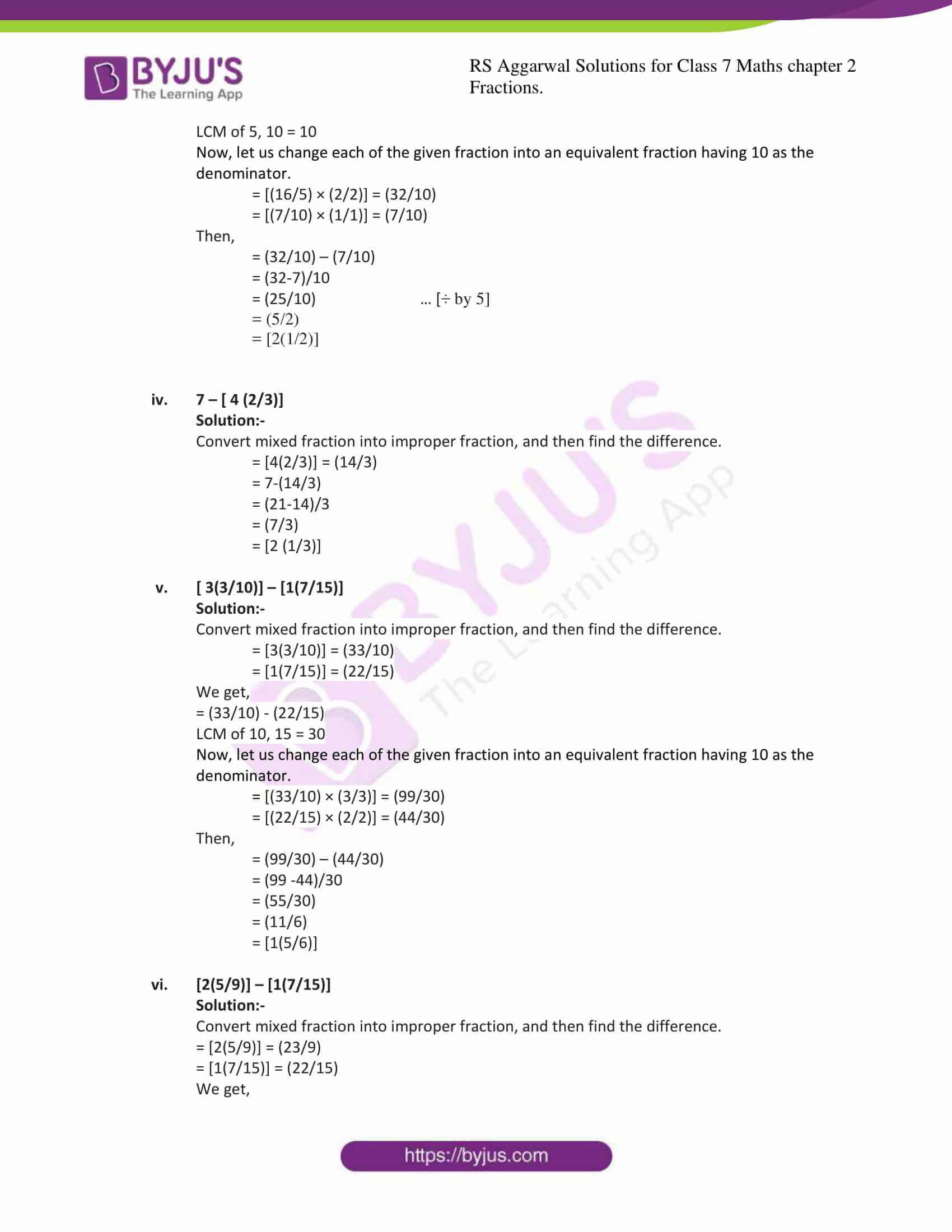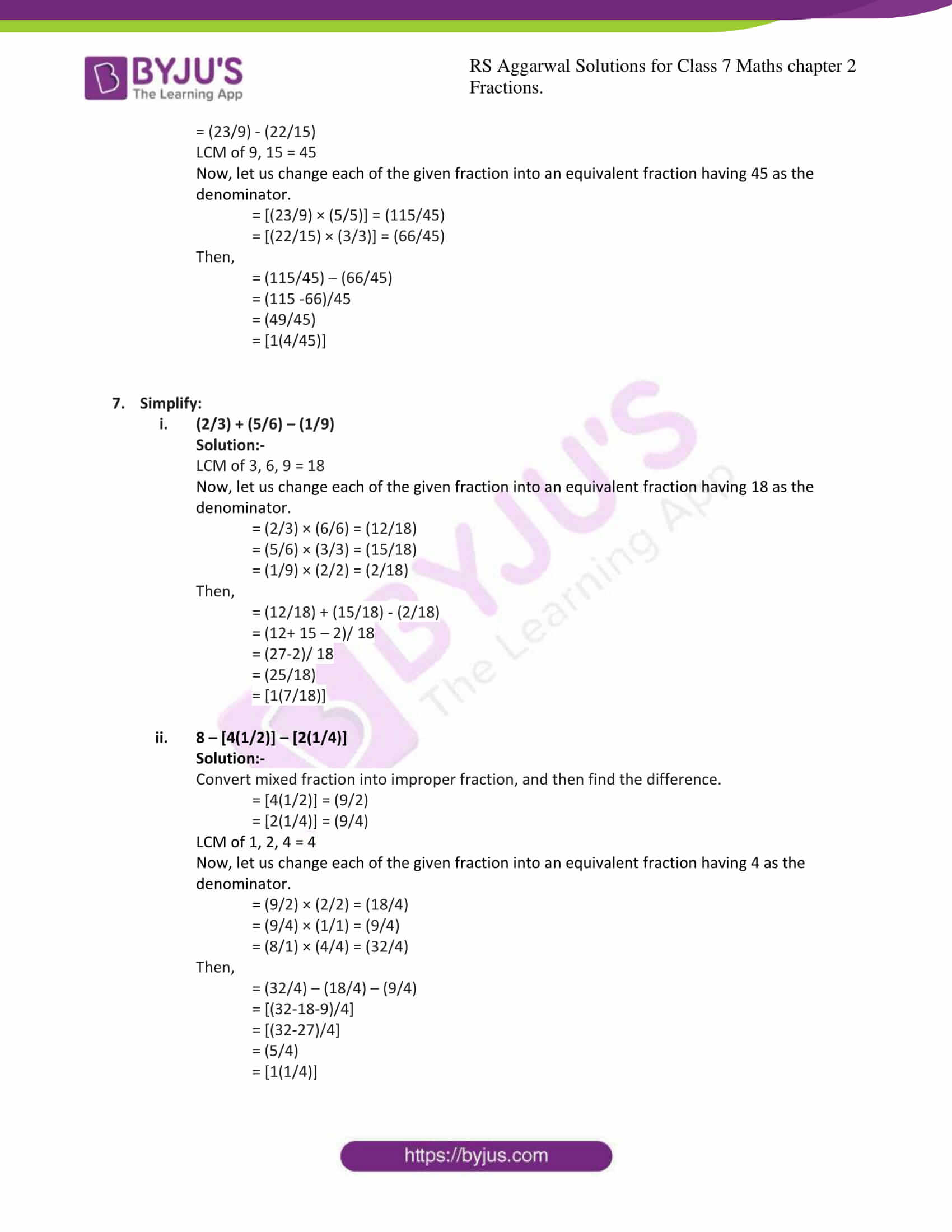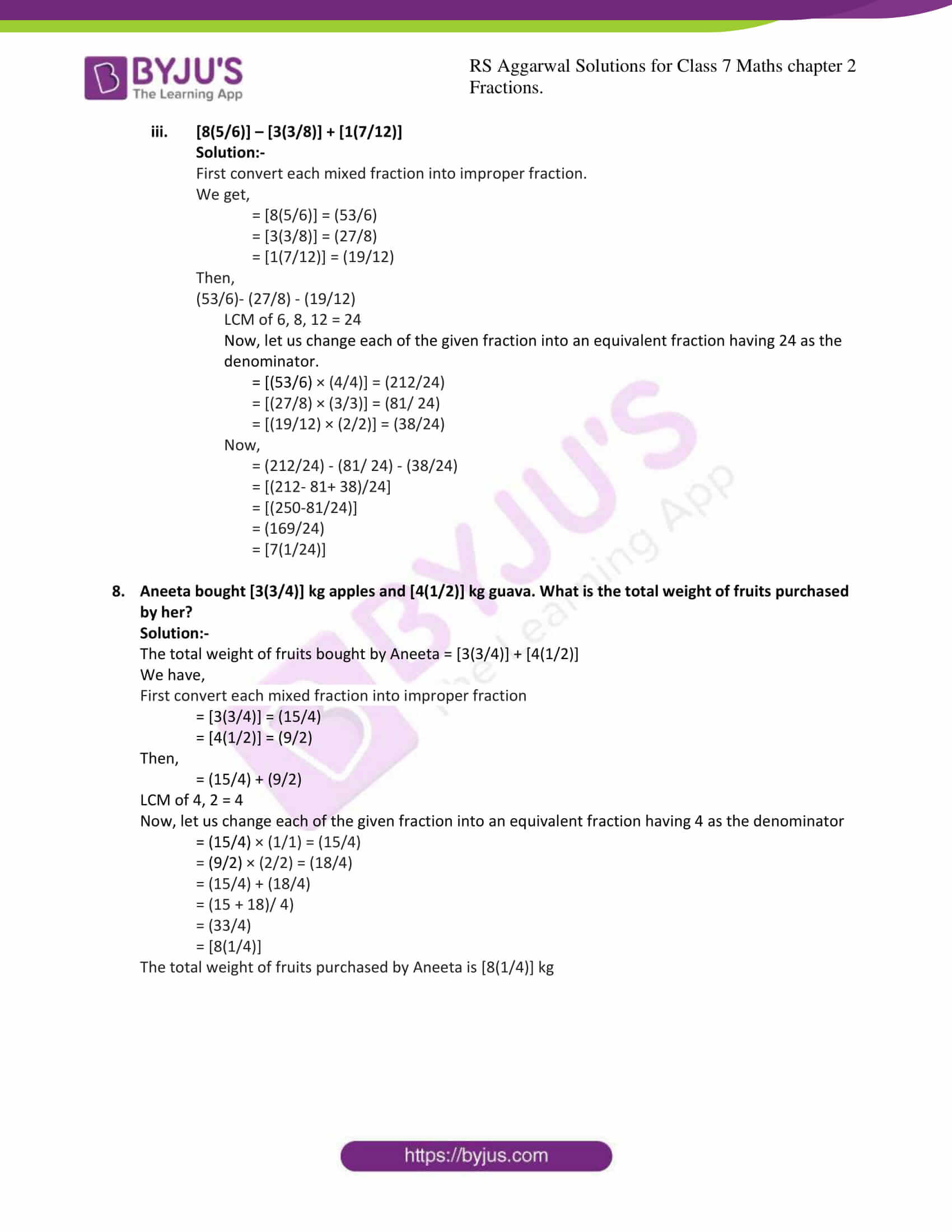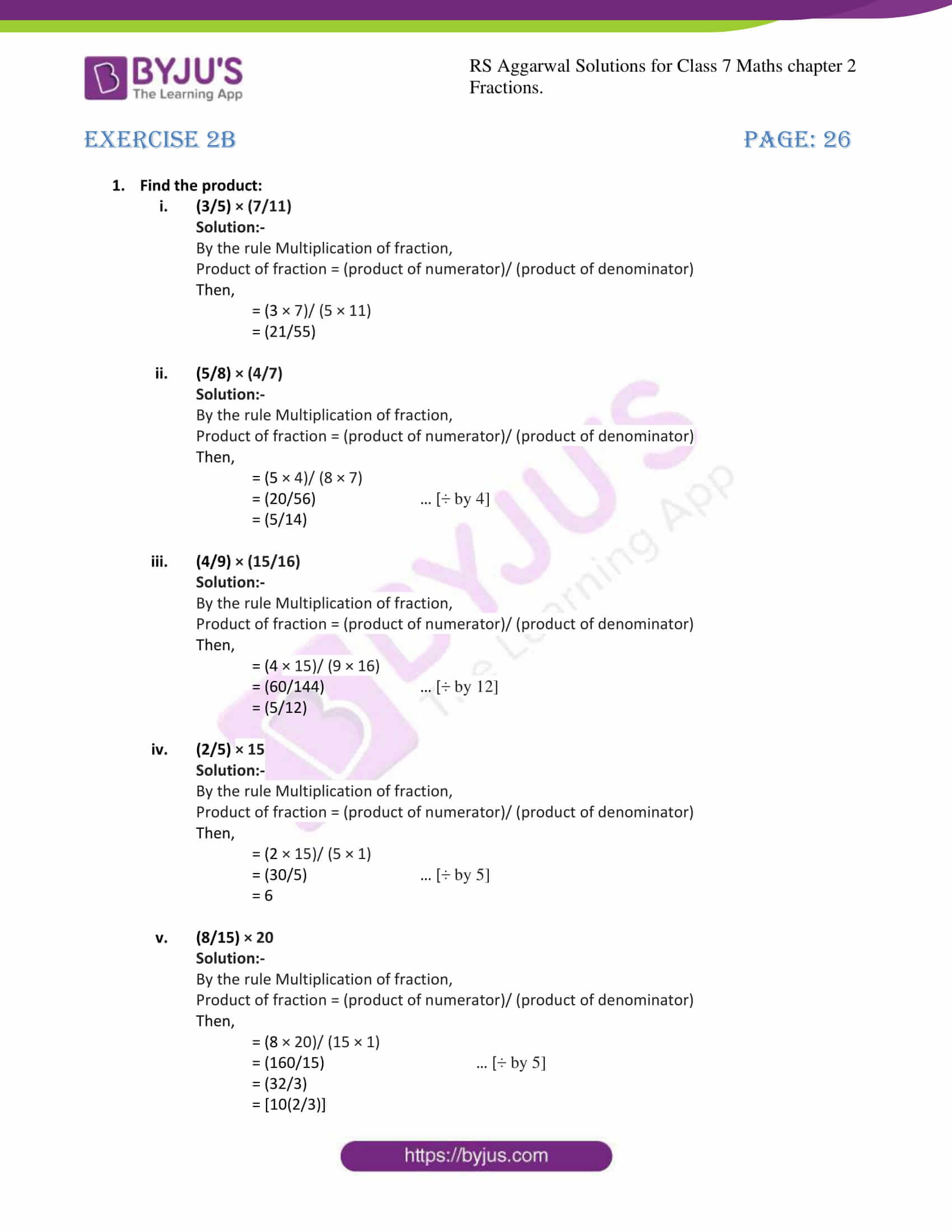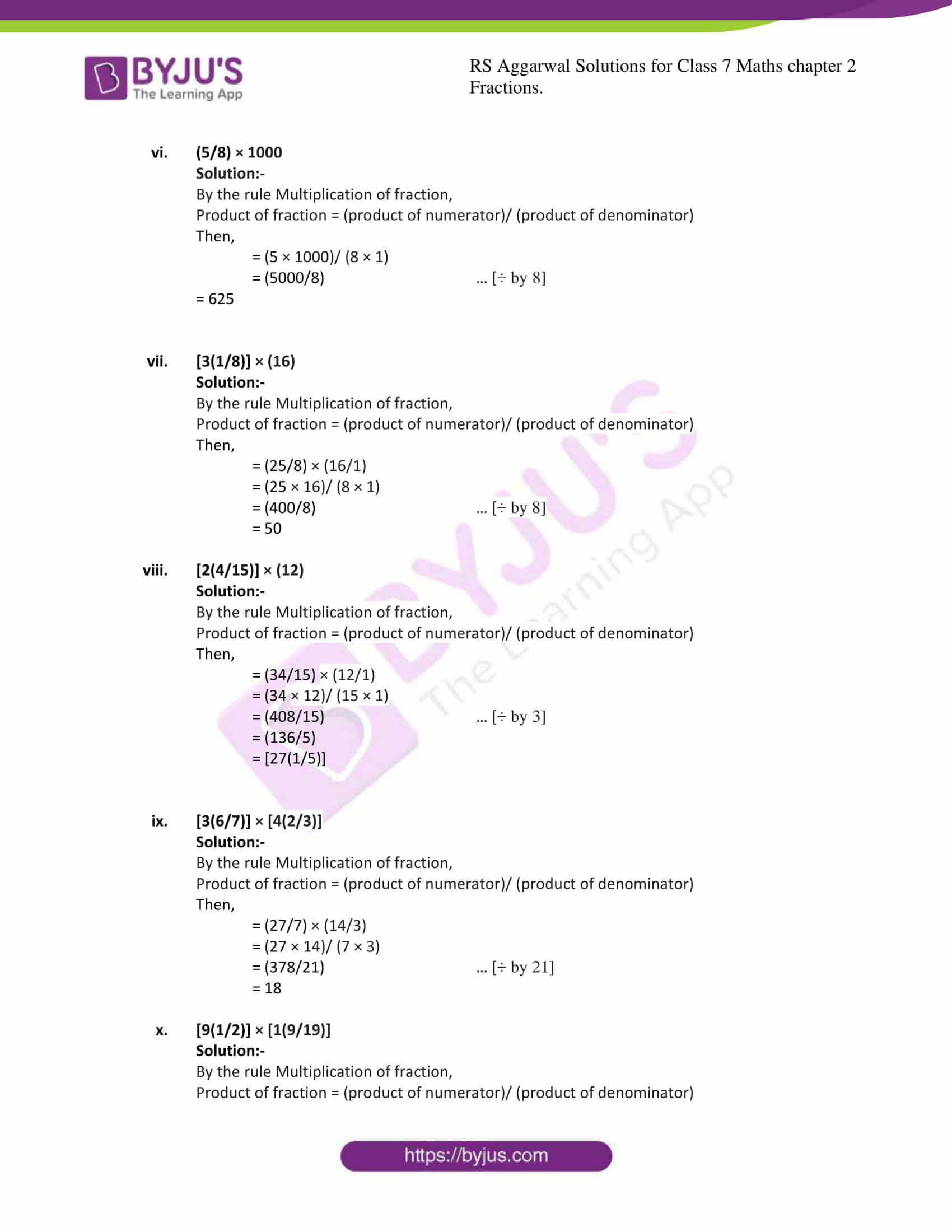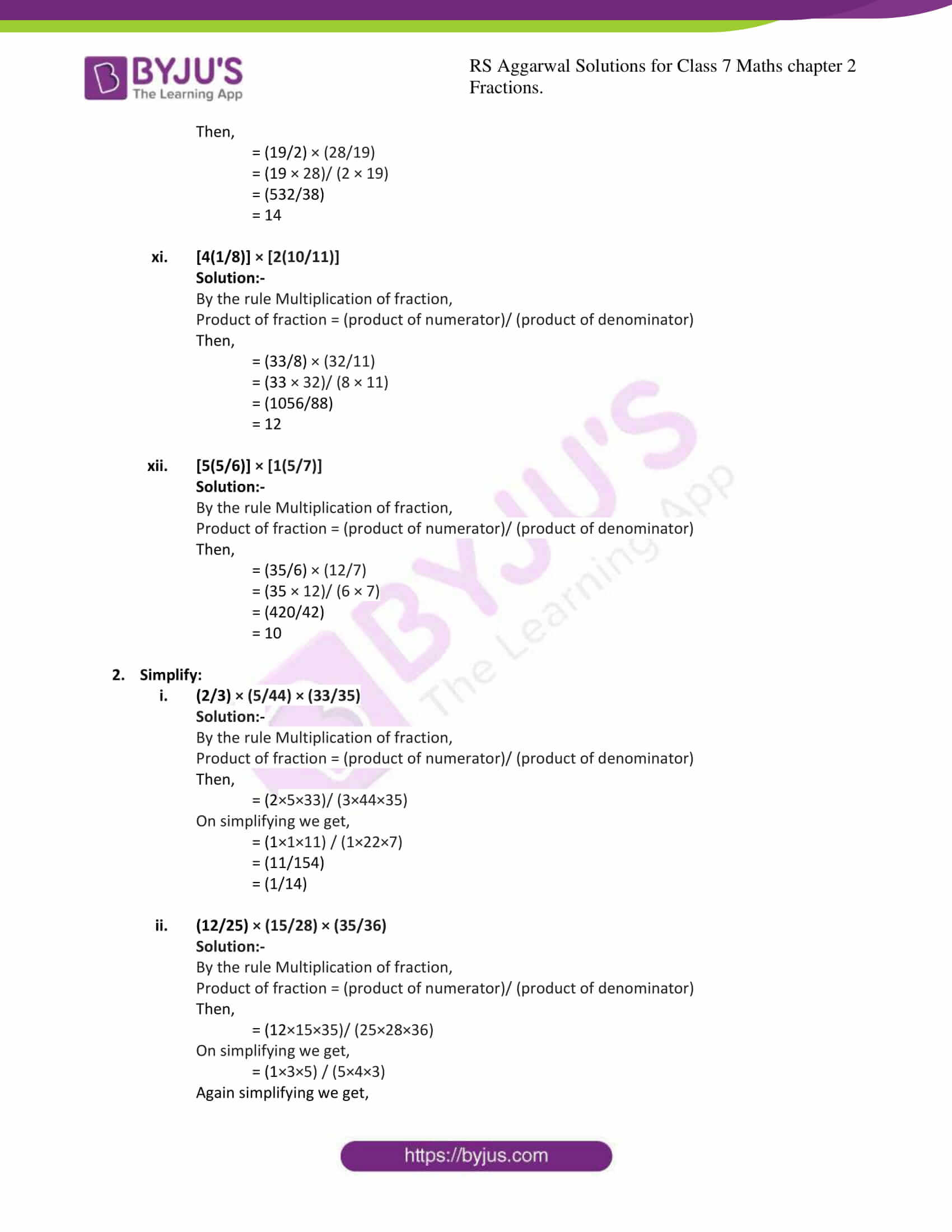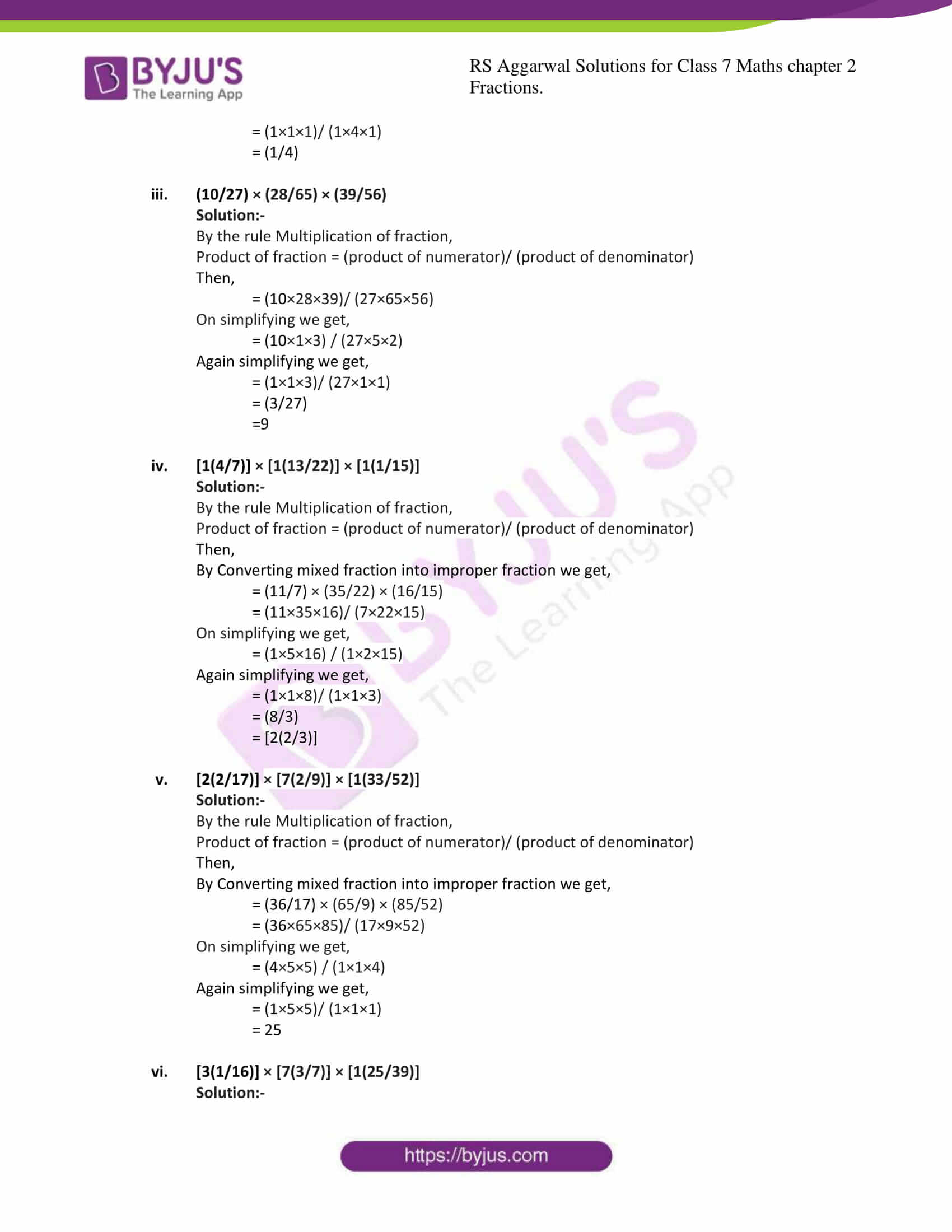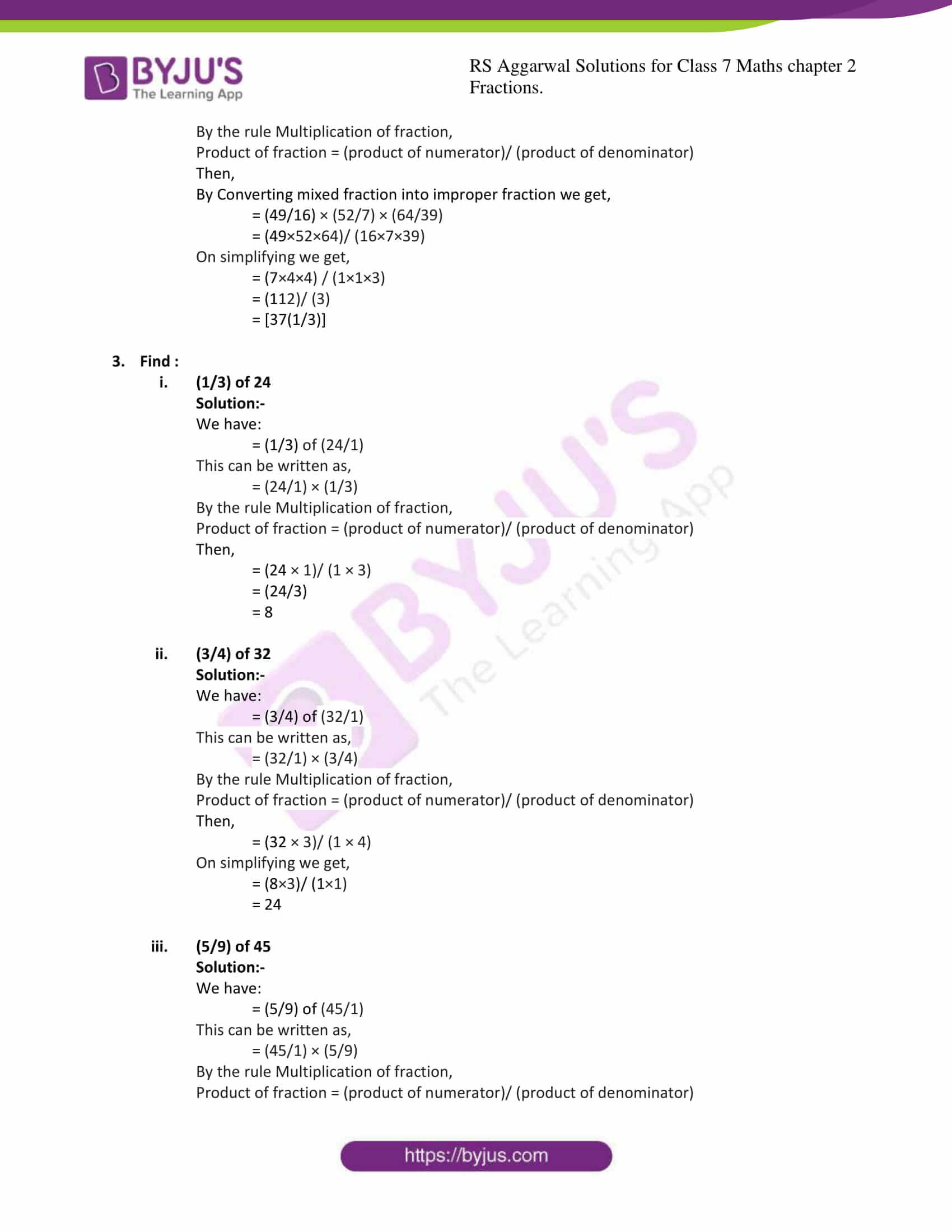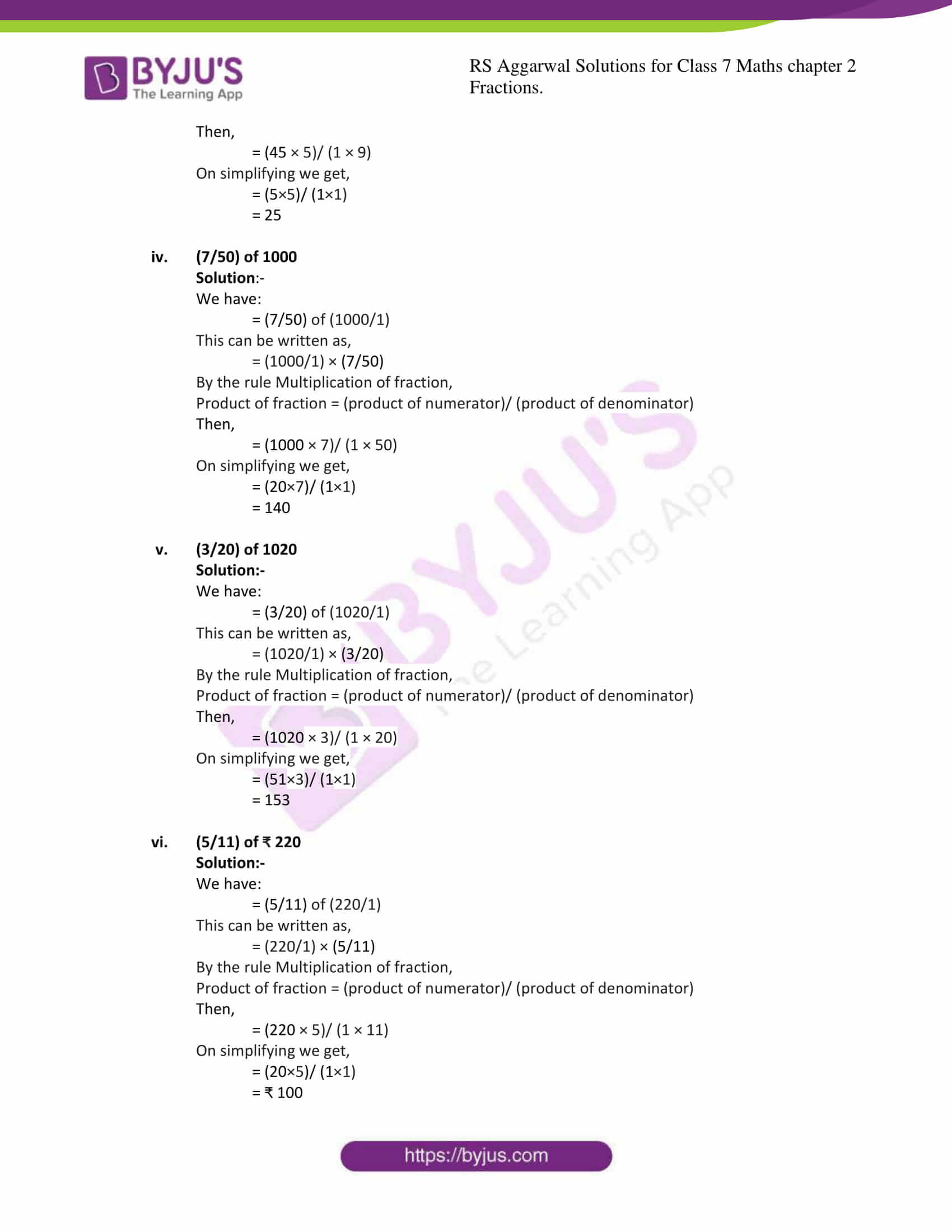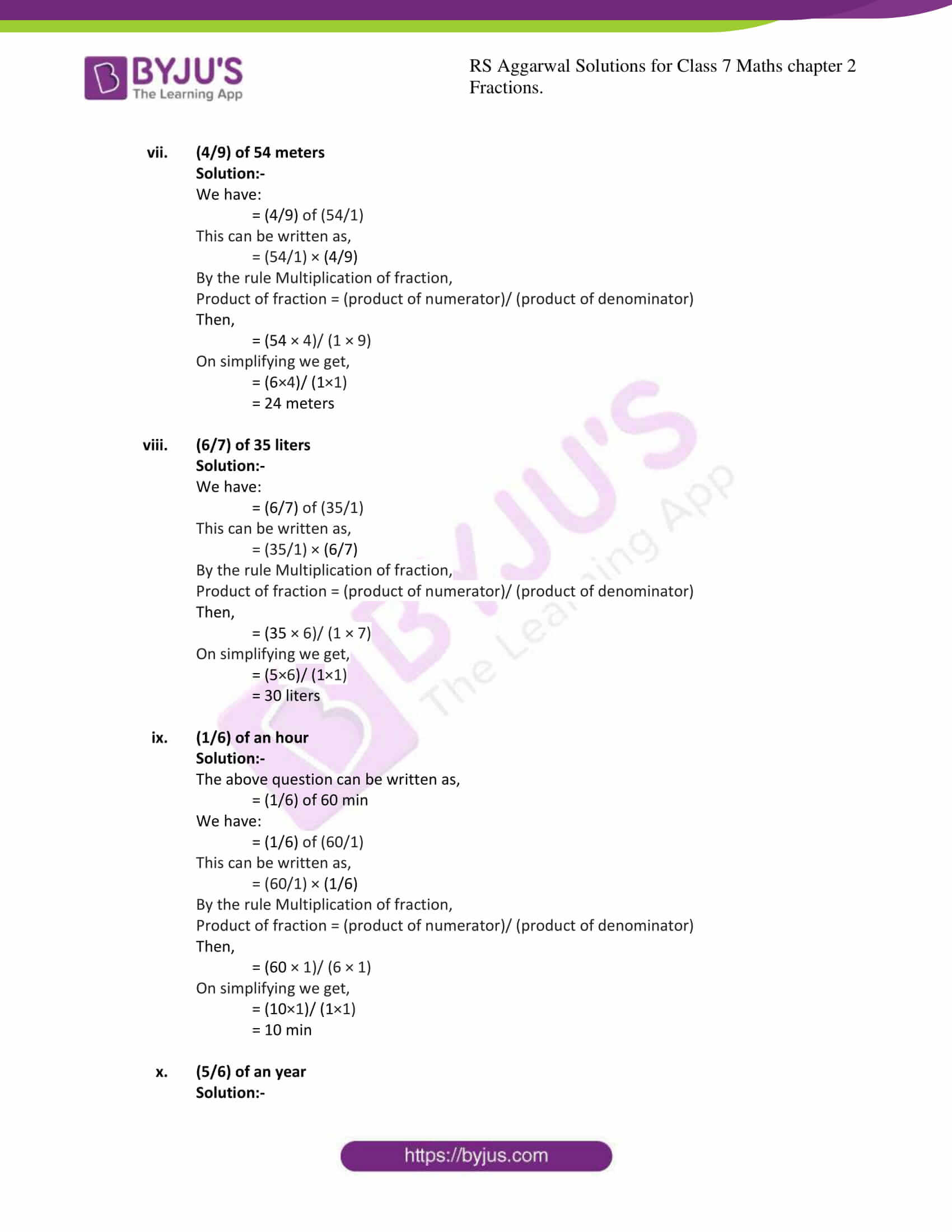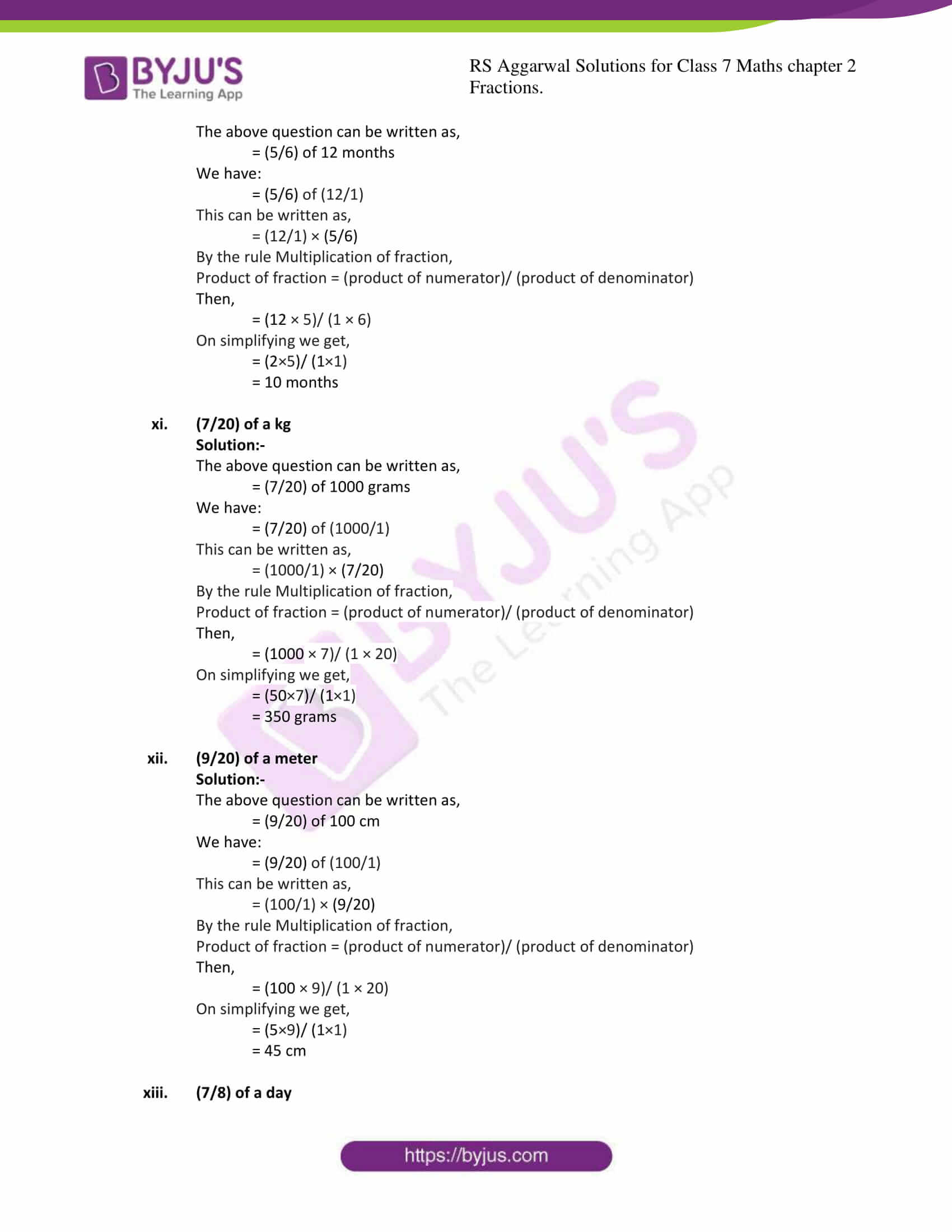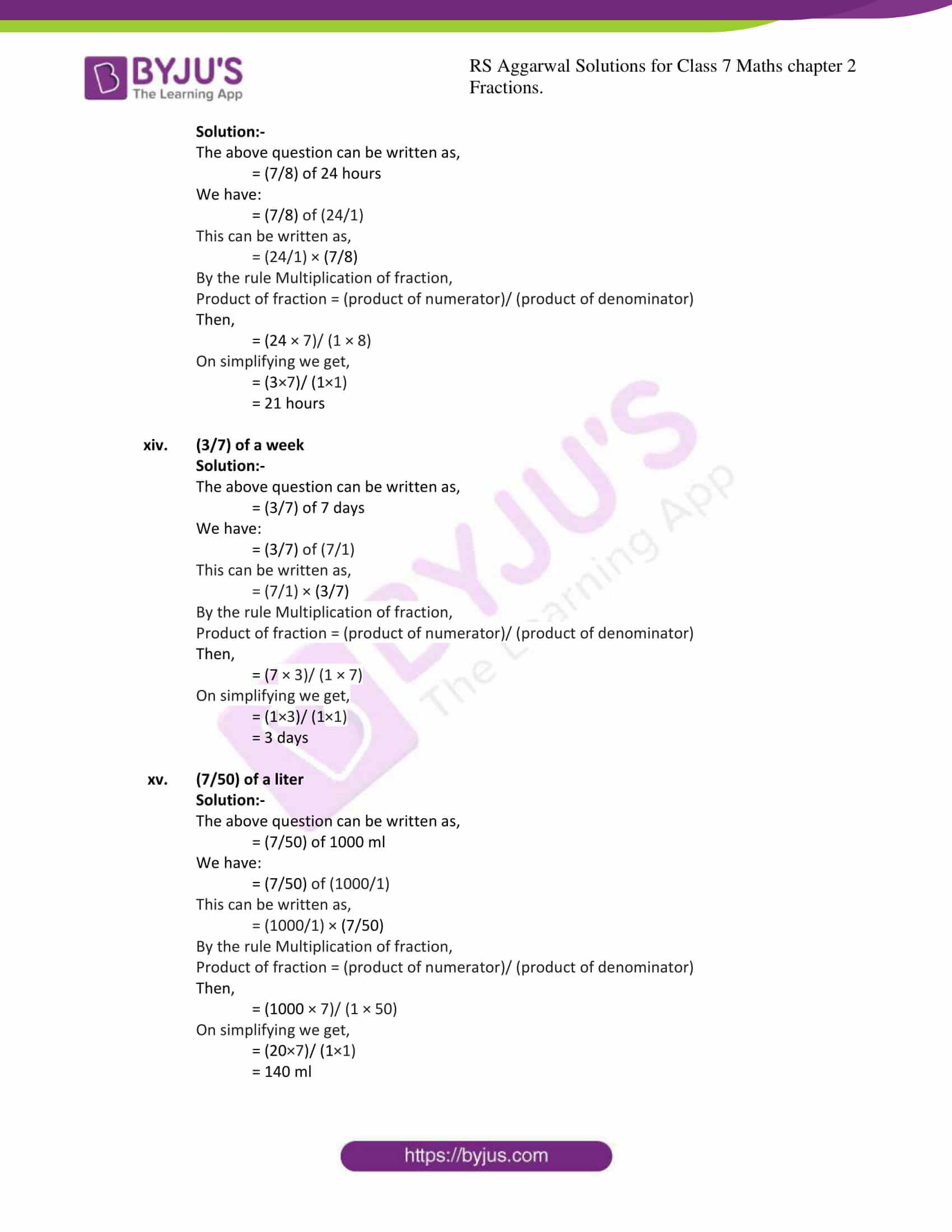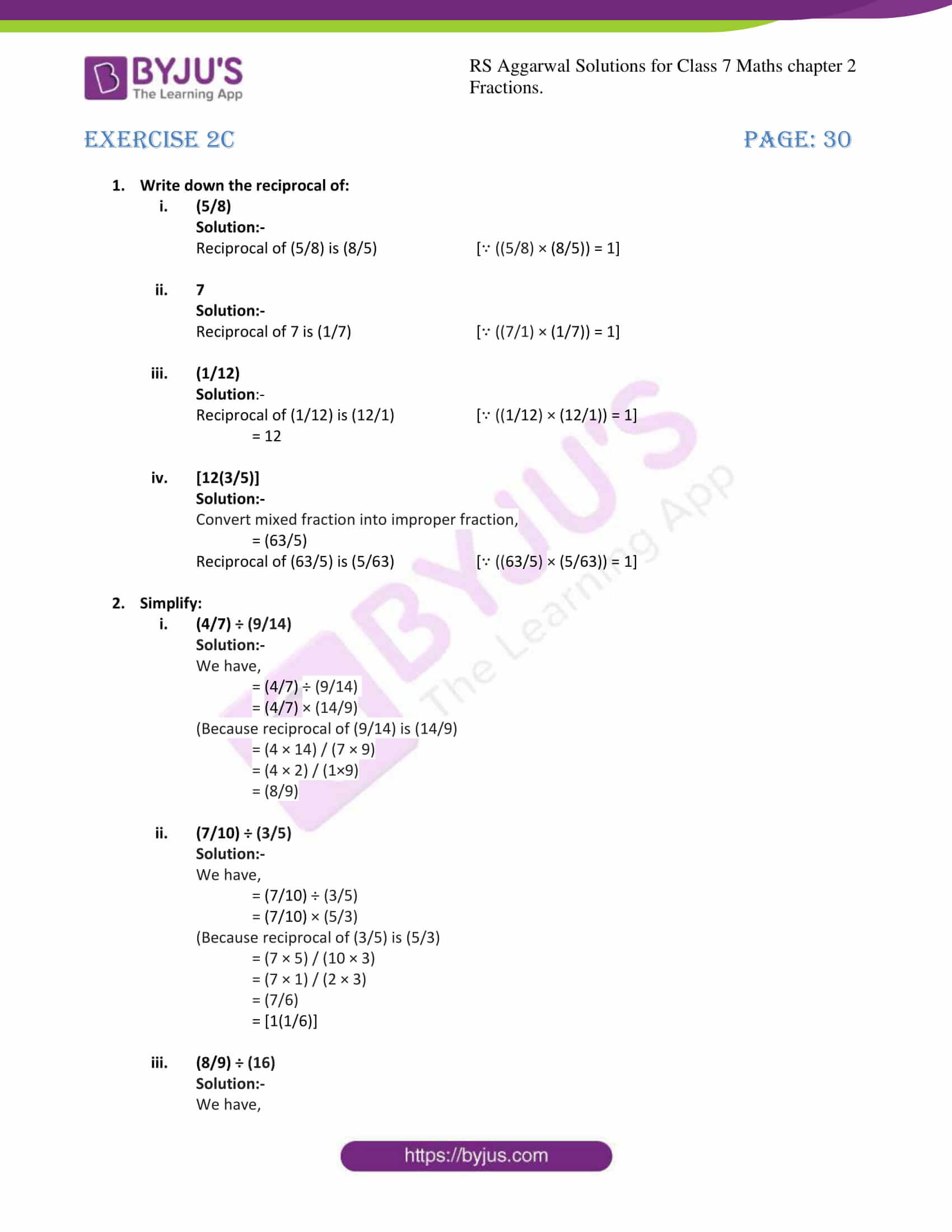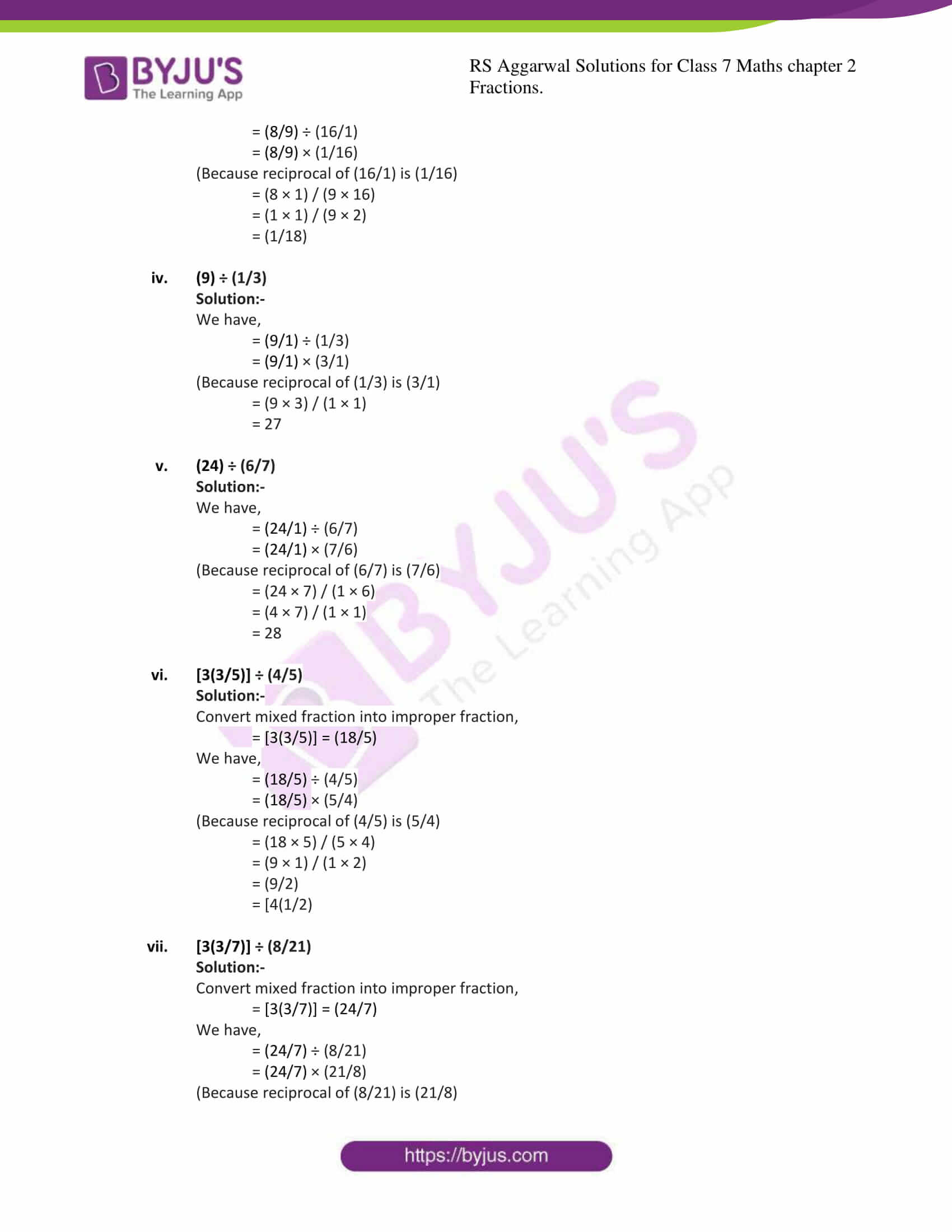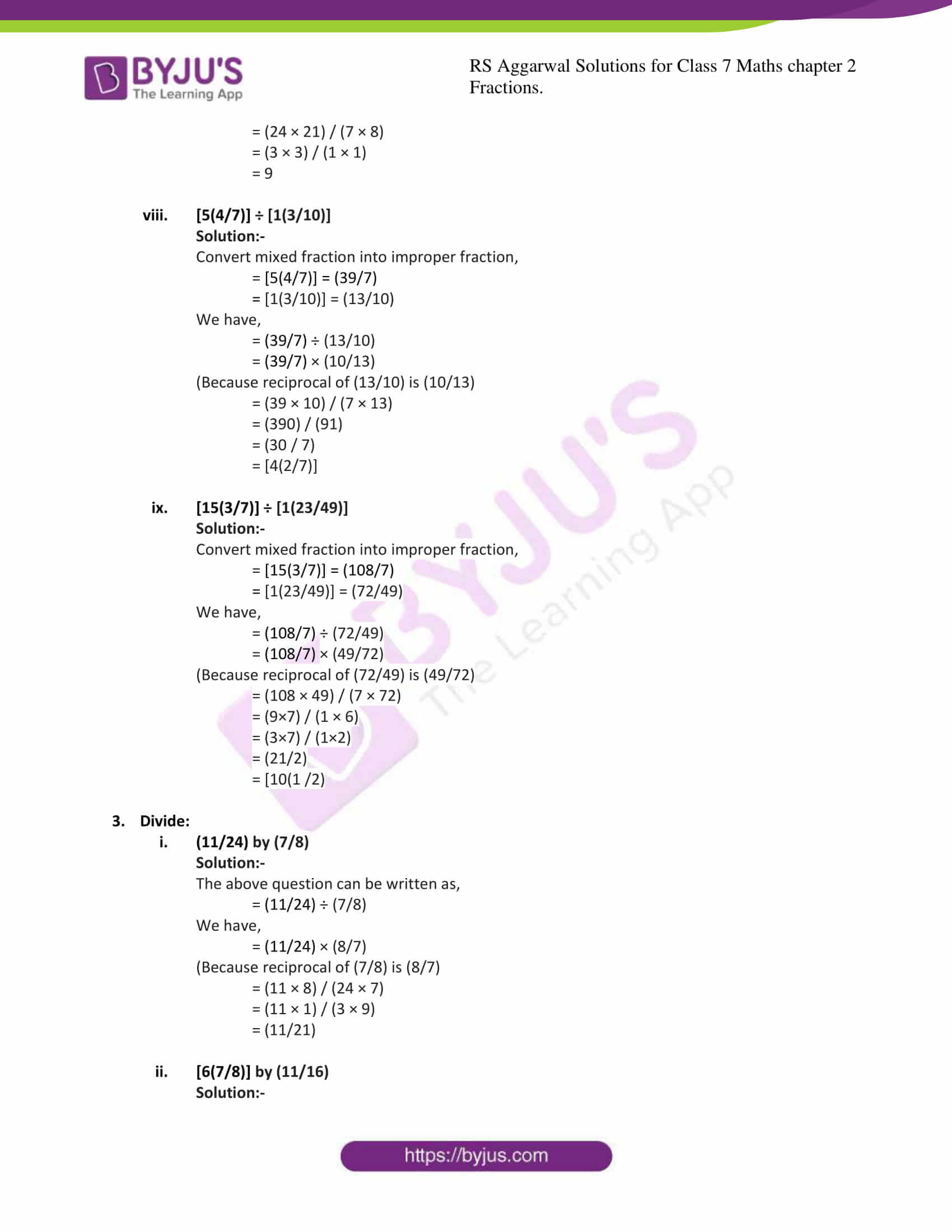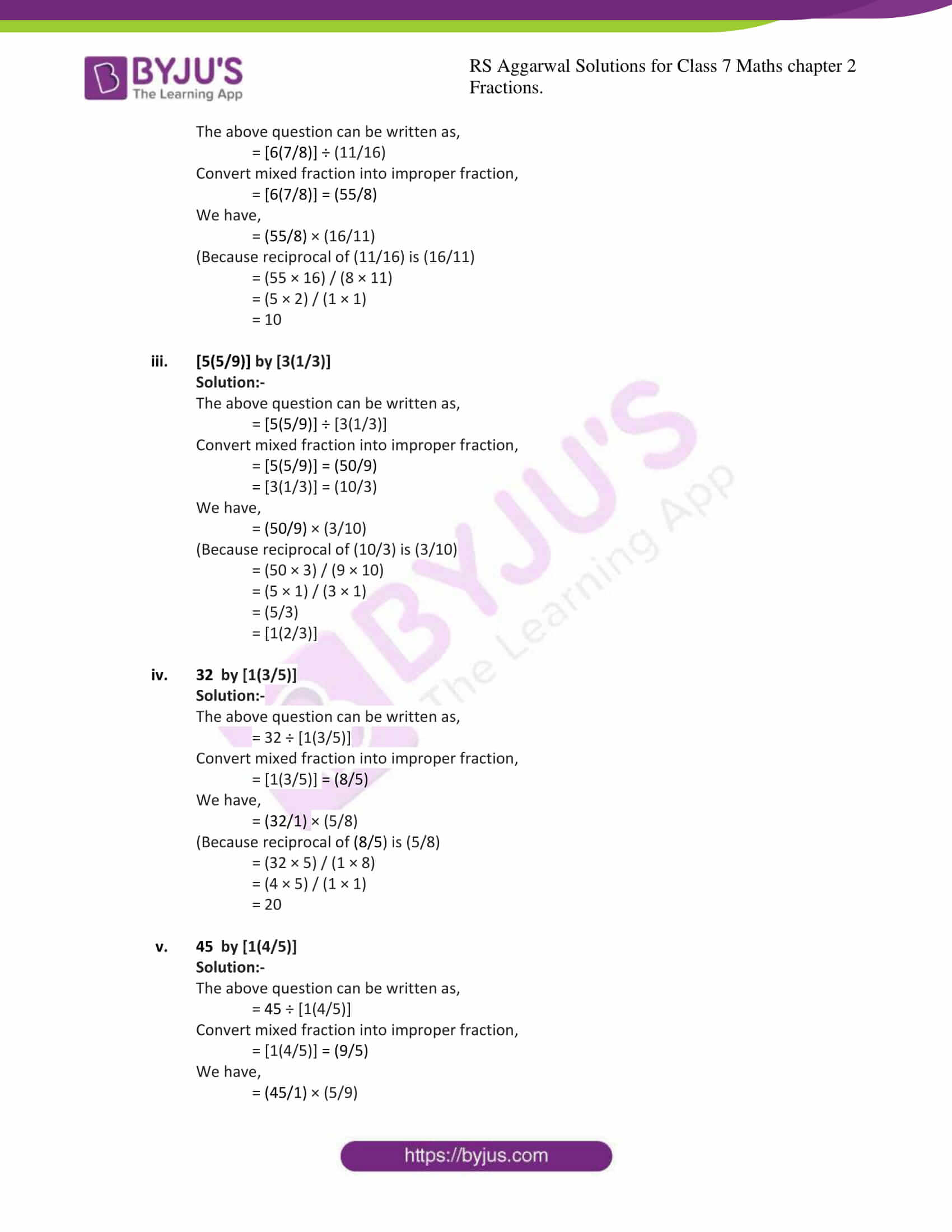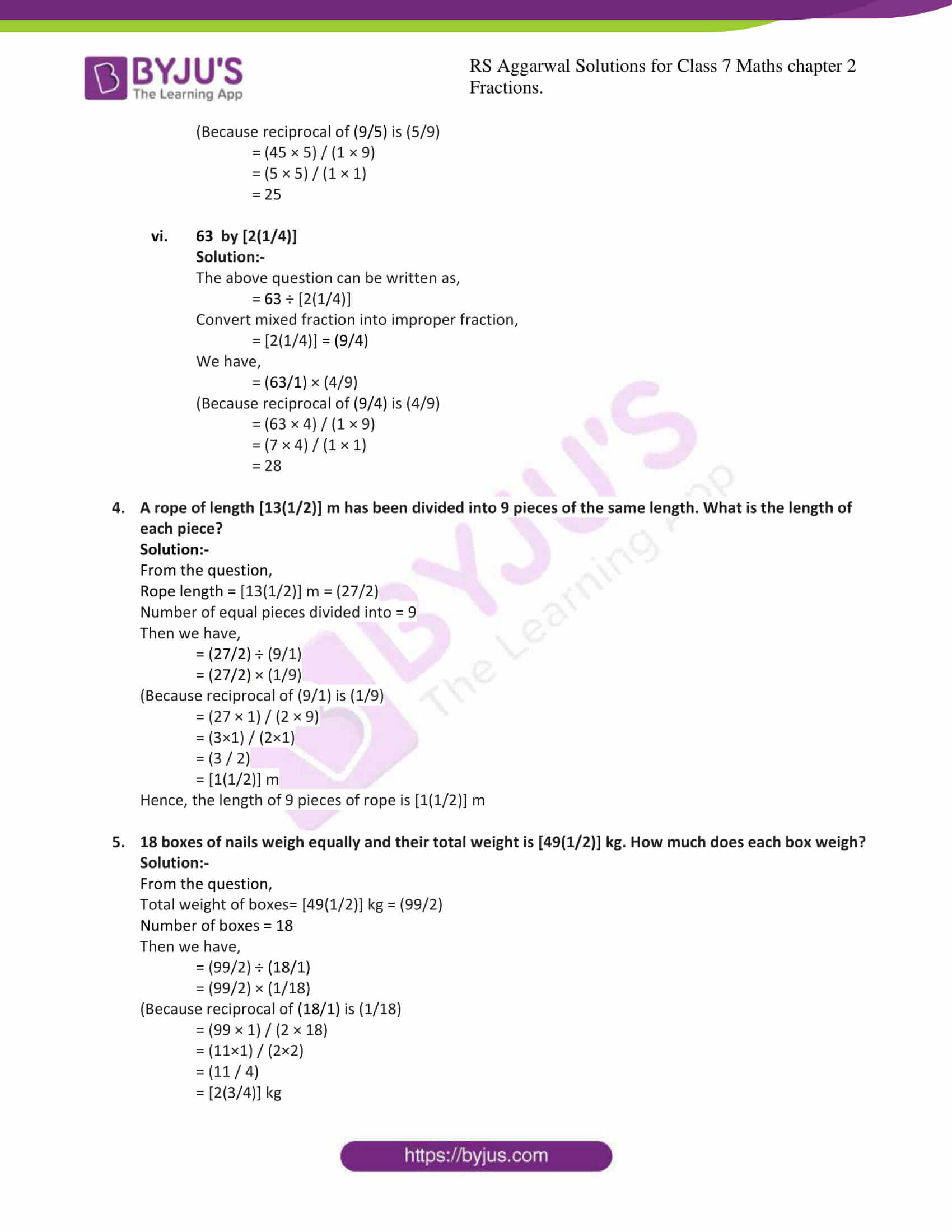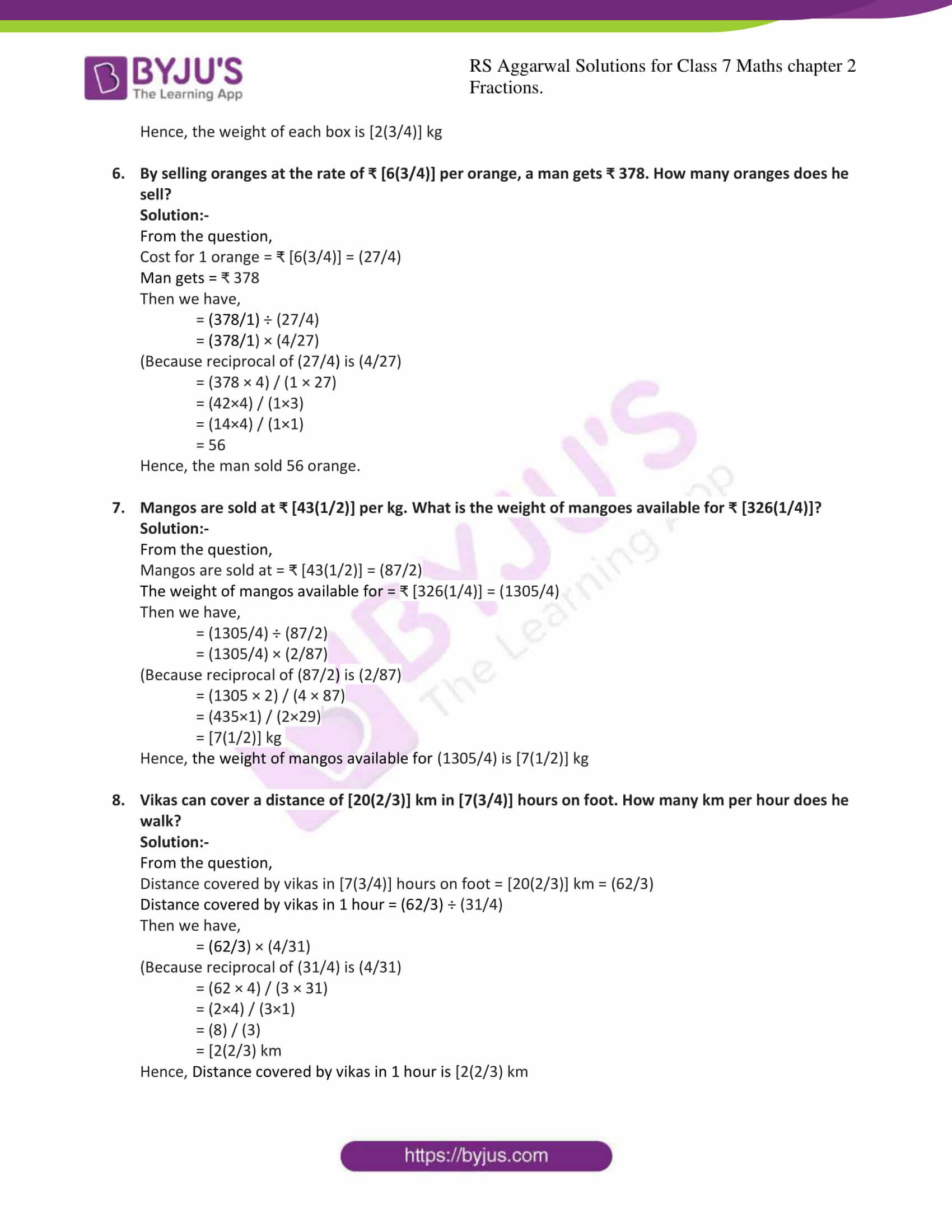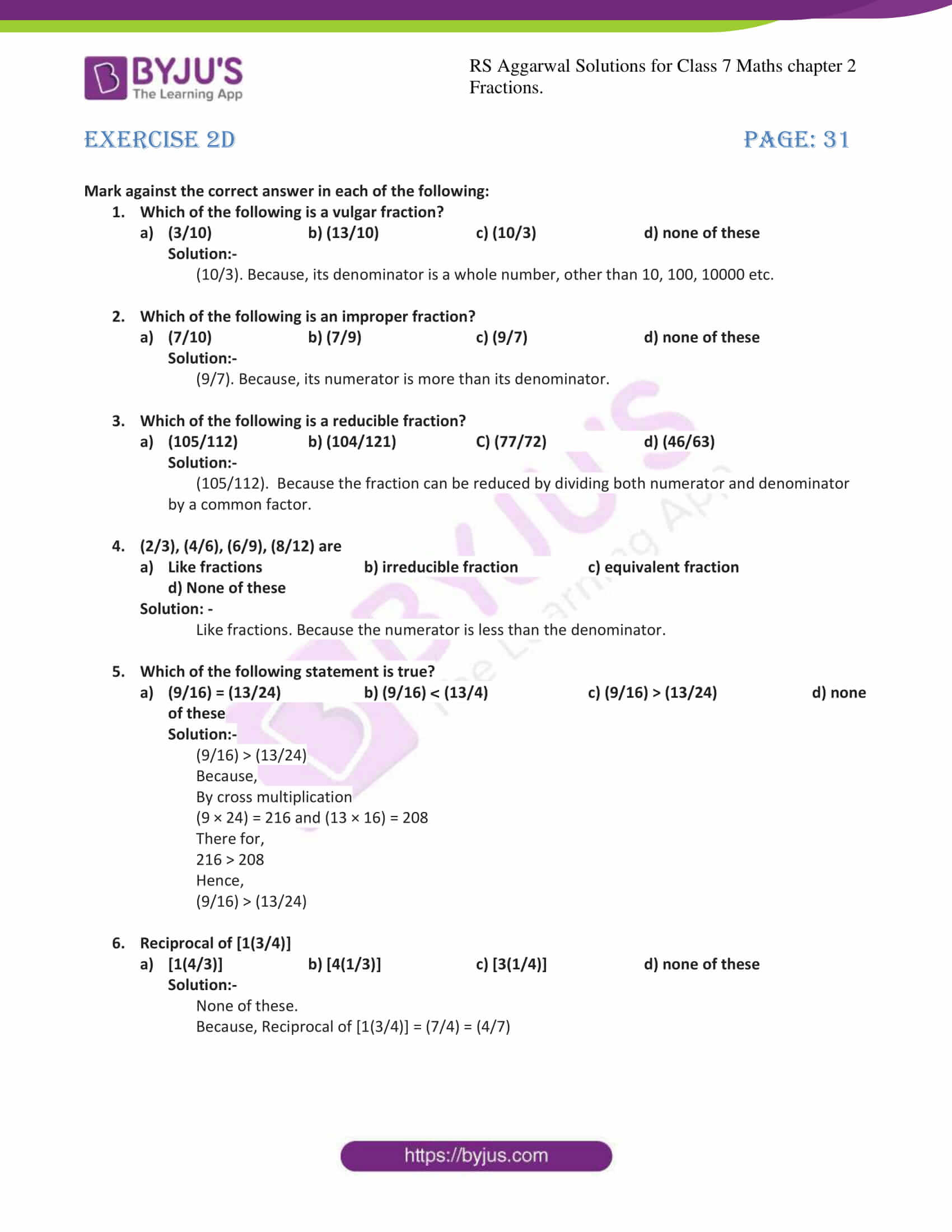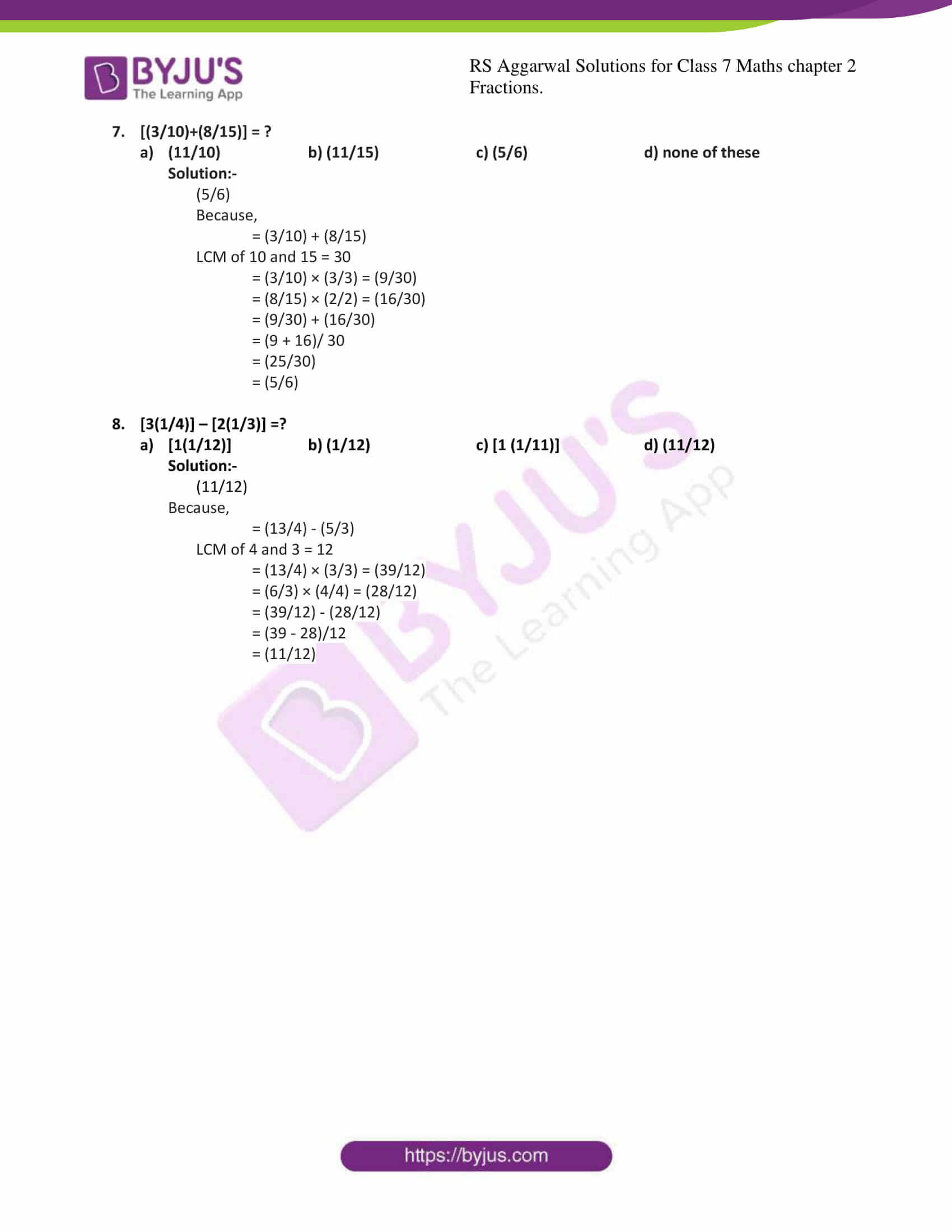Exercise 2A

Exercise 2B

Exercise 2C

Exercise 2D

## Exercise 2A

1. Compare the fraction:
(i) (5/8)and (7/12)

Solution:-

By cross multiplication, we have:

5 × 12 = 60 and 8 × 7 = 56

But,

60 > 56

∴ (5/8) > (7/12)

(ii) (5/9)and (11/15)

Solution:-

By cross multiplication, we have:

5 × 15 = 75 and 9 × 11 = 99

But,

75 < 99

∴ (5/9) < (11/15)

(iii) (11/12) and (15/16)

Solution:-

By cross multiplication, we have:

11 × 16 = 176 and 12 × 15 = 180

But,

176 < 180

∴ (11/12) < (15/16)

2. Arrange the following fraction in ascending order :
(i) (3/4), (5/6), (7/9), (11/12)

Solution:-

LCM of 4, 6, 9, 12 = 2× 2× 3× 3=36

Now, let us change each of the given fraction into an equivalent fraction having 36 as the denominator.

[(3/4) × (9/9)] = (27/36)

[(5/6) × (6/6)] = (30/36)

[(7/9) × (4/4)] = (28/360

[(11/12) × (3/3)] = (33/36)

Clearly,

(27/36) < (28/36) < (30/36) < (33/36)

Hence,

(3/4) < (7/9) < (5/6) < (11/12)

Hence, the given fractions in ascending order are (3/4), (7/9), (5/6), (11/12)

(ii) (4/5), (7/10), (11/15), (17/20)

Solution:-

LCM of 5, 10, 15, 20 = 5 × 2 × 3 × 2 = 60

Now, let us change each of the given fraction into an equivalent fraction having 60 as the denominator.

[(4/5) × (12/12)] = (48/60)

[(7/10) × (6/6)] = (42/60)

[(11/15) × (4/4)] = (44/60)

[(17/20) × (3/3)] = (52/60)

Clearly,

(42/60) < (44/60) < (48/60) < (52/60)

Hence,

(7/10) < (11/15) < (4/5) < (17/20)

Hence, the given fractions in ascending order are (7/10), (11/15), (4/5) , (17/20)

3. Arrange the following fraction in descending order :
(i) (3/4), (7/8), (7/12), (17/24)

Solution:-

LCM of 4, 8, 12, 24 = 4 × 2 × 3 = 24

Now, let us change each of the given fraction into an equivalent fraction having 24 as the denominator.

[(3/4) × (6/6)] = (18/24)

[(7/8) × (3/3)] = (21/24)

[(7/12) × (2/2)] = (14/24)

[(17/24) × (1/1)] = (17/24)

Clearly,

(21/24) > (18/24) > (17/24) > (14/24)

Hence,

(7/8) > (3/4) > (17/24) > (7/12)

Hence, the given fractions in descending order are (7/8), (3/4), (17/24), (7/12)

(ii) (2/3), (3/5), (7/10), (8/15)

Solution:-

LCM of 3, 5, 10, 15 = 3 × 5 × 2 = 30

Now, let us change each of the given fraction into an equivalent fraction having 30 as the denominator.

[(2/3) × (10/10)] = (20/30)

[(3/5) × (6/6)]= (18/30)

[(7/10) × (3/3) = (21/30)

[(8/15) × (2/2)] = (16/30)

Clearly,

(21/30) > (20/30) > (18/30) > (16/30)

Hence,

(7/10) > (2/3) > (3/5) > (8/15)

Hence, the given fractions in descending order are (7/10), (2/3), (3/5), (8/15)

4. Reenu got (2/7) part of an apple while sonal got (4/5) part of it. Who got the larger part and by how much?

Solution:-

From the question,

Reenu got (2/7) part of an apple

Sonal got (4/5) part of an apple

First we have to compare the given fraction (2/7) and (4/5) to know who got the larger part of the apple.

Then,

By cross multiplication, we have

2 × 5 = 10 and 4 × 7 = 28

But,

10 < 28

∴ (2/7) < (4/5)

So, Sonal got the larger part of the apple

Now,

= (4/5)-(2/7)

= [(28-10)/35]

= [18/35]

∴ Sonal got (18/35) part of the apple larger than Reenu.

5. Find the sum:
(i) (5/9)+(3/9)

Solution:-

For adding two like fractions, the numerators are added and the denominator remains the same.

= (5+3)/9

= (8/9)

(ii) (8/9) + (7/12)

Solution:-

For addition of two unlike fractions, first change them to the like fractions.

LCM of 9, 12 = 36

Now, let us change each of the given fraction into an equivalent fraction having 36 as the denominator.

[(8/9) × (4/4)] = (32/36)

[(7/12) × (3/3)] = (21/36)

= (32/36) + (21/36)

= (32+21)/36

= (53/36)

= [1(17/36)]

(iii) [3(4/5)] + [2(3/10)] + [1(1/15)]

Solution:-

First convert each mixed fraction into improper fraction.

We get,

= [3(4/5)] = (18/5)

= [2(3/10)] = (23/10)

= [1(1/15)] = (16/15)

Then,

(19/5) + (23/10) + (16/15)

LCM of 5, 10, 15 = 30

Now, let us change each of the given fraction into an equivalent fraction having 30 as the denominator.

= [(19/5) × (6/6)] = (114/30)

= [(23/10) × (3/3)] = (69/ 30)

= [(16/15) × (2/2)] = (32/30)

Now,

= (114/30) + (69/30) + (32/30)

= [(114+69+32)/30]

= (215/30)

= [7 (5/30)]

= [7 (1/5)]

6. Find the difference:
(i) (5/7) – (2/7)

Solution:-

The subtraction of fraction can be performed in a manner similar to that of addition.

For subtracting two like fractions, the numerators are subtract and the denominator remains the same.

= (5 – 2)/7

= (3/7)

(ii) (5/6) – (3/4)

Solution:-

For subtraction of two unlike fractions, first change them to the like fractions.

LCM of 6, 4 = 12

Now, let us change each of the given fraction into an equivalent fraction having 12 as the denominator.

= [(5/6) × (2/2)] = (10/12)

= [(3/4) × (3/3)] = (9/12)

Now,

= (10/12)-(9/12)

= [(10 – 9)/12]

= (1/12)

(iii) [3(1/5)] – (7/10)

Solution:-

Convert mixed fraction into improper fraction,

= [3(1/5)] = (16/5)

= (16/5)-(7/10)

For subtraction of two unlike fractions, first change them to the like fractions.

LCM of 5, 10 = 10

Now, let us change each of the given fraction into an equivalent fraction having 10 as the denominator.

= [(16/5) × (2/2)] = (32/10)

= [(7/10) × (1/1)] = (7/10)

Then,

= (32/10) – (7/10)

= (32-7)/10

= (25/10) … [÷ by 5]

= (5/2)

= [2(1/2)]

(iv) 7 – [ 4 (2/3)]

Solution:-

Convert mixed fraction into improper fraction, and then find the difference.

= [4(2/3)] = (14/3)

= 7-(14/3)

= (21-14)/3

= (7/3)

= [2 (1/3)]

(v) [ 3(3/10)] – [1(7/15)]

Solution:-

Convert mixed fraction into improper fraction, and then find the difference.

= [3(3/10)] = (33/10)

= [1(7/15)] = (22/15)

We get,

= (33/10) – (22/15)

LCM of 10, 15 = 30

Now, let us change each of the given fraction into an equivalent fraction having 10 as the denominator.

= [(33/10) × (3/3)] = (99/30)

= [(22/15) × (2/2)] = (44/30)

Then,

= (99/30) – (44/30)

= (99 -44)/30

= (55/30)

= (11/6)

= [1(5/6)]

(vi) [2(5/9)] – [1(7/15)]

Solution:-

Convert mixed fraction into improper fraction, and then find the difference.

= [2(5/9)] = (23/9)

= [1(7/15)] = (22/15)

We get,

= (23/9) – (22/15)

LCM of 9, 15 = 45

Now, let us change each of the given fraction into an equivalent fraction having 45 as the denominator.

= [(23/9) × (5/5)] = (115/45)

= [(22/15) × (3/3)] = (66/45)

Then,

= (115/45) – (66/45)

= (115 -66)/45

= (49/45)

= [1(4/45)]

7. Simplify:
(i) (2/3) + (5/6) – (1/9)

Solution:-

LCM of 3, 6, 9 = 18

Now, let us change each of the given fraction into an equivalent fraction having 18 as the denominator.

= (2/3) × (6/6) = (12/18)

= (5/6) × (3/3) = (15/18)

= (1/9) × (2/2) = (2/18)

Then,

= (12/18) + (15/18) – (2/18)

= (12+ 15 – 2)/ 18

= (27-2)/ 18

= (25/18)

= [1(7/18)]

(ii) 8 – [4(1/2)] – [2(1/4)]

Solution:-

Convert mixed fraction into improper fraction, and then find the difference.

= [4(1/2)] = (9/2)

= [2(1/4)] = (9/4)

LCM of 1, 2, 4 = 4

Now, let us change each of the given fraction into an equivalent fraction having 4 as the denominator.

= (9/2) × (2/2) = (18/4)

= (9/4) × (1/1) = (9/4)

= (8/1) × (4/4) = (32/4)

Then,

= (32/4) – (18/4) – (9/4)

= [(32-18-9)/4]

= [(32-27)/4]

= (5/4)

= [1(1/4)]

(iii) [8(5/6)] – [3(3/8)] + [1(7/12)]

Solution:-

First convert each mixed fraction into improper fraction.

We get,

= [8(5/6)] = (53/6)

= [3(3/8)] = (27/8)

= [1(7/12)] = (19/12)

Then,

(53/6)- (27/8) – (19/12)

LCM of 6, 8, 12 = 24

Now, let us change each of the given fraction into an equivalent fraction having 24 as the denominator.

= [(53/6) × (4/4)] = (212/24)

= [(27/8) × (3/3)] = (81/ 24)

= [(19/12) × (2/2)] = (38/24)

Now,

= (212/24) – (81/ 24) – (38/24)

= [(212- 81+ 38)/24]

= [(250-81/24)]

= (169/24)

= [7(1/24)]

8. Aneeta bought [3(3/4)] kg apples and [4(1/2)] kg guava. What is the total weight of fruits purchased by her?

Solution:-

The total weight of fruits bought by Aneeta = [3(3/4)] + [4(1/2)]

We have,

First convert each mixed fraction into improper fraction

= [3(3/4)] = (15/4)

= [4(1/2)] = (9/2)

Then,

= (15/4) + (9/2)

LCM of 4, 2 = 4

Now, let us change each of the given fraction into an equivalent fraction having 4 as the denominator

= (15/4) × (1/1) = (15/4)

= (9/2) × (2/2) = (18/4)

= (15/4) + (18/4)

= (15 + 18)/ 4)

= (33/4)

= [8(1/4)]

The total weight of fruits purchased by Aneeta is [8(1/4)] kg

## Exercise 2B

1. Find the product:
(i) (3/5) × (7/11)

Solution:-

By the rule Multiplication of fraction,

Product of fraction = (product of numerator)/ (product of denominator)

Then,

= (3 × 7)/ (5 × 11)

= (21/55)

(ii) (5/8) × (4/7)

Solution:-

By the rule Multiplication of fraction,

Product of fraction = (product of numerator)/ (product of denominator)

Then,

= (5 × 4)/ (8 × 7)

= (20/56) … [÷ by 4]

= (5/14)

(iii) (4/9) × (15/16)

Solution:-

By the rule Multiplication of fraction,

Product of fraction = (product of numerator)/ (product of denominator)

Then,

= (4 × 15)/ (9 × 16)

= (60/144) … [ by 12]

= (5/12)

(iv) (2/5) × 15

Solution:-

By the rule Multiplication of fraction,

Product of fraction = (product of numerator)/ (product of denominator)

Then,

= (2 × 15)/ (5 × 1)

= (30/5) … [÷ by 5]

= 6

(v) (8/15) × 20

Solution:-

By the rule Multiplication of fraction,

Product of fraction = (product of numerator)/ (product of denominator)

Then,

= (8 × 20)/ (15 × 1)

= (160/15) … [÷ by 5]

= (32/3)

= [10(2/3)]

(vi) (5/8) × 1000

Solution:-

By the rule Multiplication of fraction,

Product of fraction = (product of numerator)/ (product of denominator)

Then,

= (5 × 1000)/ (8 × 1)

= (5000/8) … [÷ by 8]

= 625

(vii)[3(1/8)] × (16)

Solution:-

By the rule Multiplication of fraction,

Product of fraction = (product of numerator)/ (product of denominator)

Then,

= (25/8) × (16/1)

= (25 × 16)/ (8 × 1)

= (400/8) … [÷ by 8]

= 50

(viii)[2(4/15)] × (12)

Solution:-

By the rule Multiplication of fraction,

Product of fraction = (product of numerator)/ (product of denominator)

Then,

= (34/15) × (12/1)

= (34 × 12)/ (15 × 1)

= (408/15) … [÷ by 3]

= (136/5)

= [27(1/5)]

(ix)[3(6/7)] × [4(2/3)]

Solution:-

By the rule Multiplication of fraction,

Product of fraction = (product of numerator)/ (product of denominator)

Then,

= (27/7) × (14/3)

= (27 × 14)/ (7 × 3)

= (378/21) … [÷ by 21]

= 18

(x) [9(1/2)] × [1(9/19)]

Solution:-

By the rule Multiplication of fraction,

Product of fraction = (product of numerator)/ (product of denominator)

Then,

= (19/2) × (28/19)

= (19 × 28)/ (2 × 19)

= (532/38)

= 14

(xi) [4(1/8)] × [2(10/11)]

Solution:-

By the rule Multiplication of fraction,

Product of fraction = (product of numerator)/ (product of denominator)

Then,

= (33/8) × (32/11)

= (33 × 32)/ (8 × 11)

= (1056/88)

= 12

(xii) [5(5/6)] × [1(5/7)]

Solution:-

By the rule Multiplication of fraction,

Product of fraction = (product of numerator)/ (product of denominator)

Then,

= (35/6) × (12/7)

= (35 × 12)/ (6 × 7)

= (420/42)

= 10

2. Simplify:
(i) (2/3) × (5/44) × (33/35)

Solution:-

By the rule Multiplication of fraction,

Product of fraction = (product of numerator)/ (product of denominator)

Then,

= (2×5×33)/ (3×44×35)

On simplifying we get,

= (1×1×11) / (1×22×7)

= (11/154)

= (1/14)

(ii) (12/25) × (15/28) × (35/36)

Solution:-

By the rule Multiplication of fraction,

Product of fraction = (product of numerator)/ (product of denominator)

Then,

= (12×15×35)/ (25×28×36)

On simplifying we get,

= (1×3×5) / (5×4×3)

Again simplifying we get,

= (1×1×1)/ (1×4×1)

= (1/4)

(iii) (10/27) × (28/65) × (39/56)

Solution:-

By the rule Multiplication of fraction,

Product of fraction = (product of numerator)/ (product of denominator)

Then,

= (10×28×39)/ (27×65×56)

On simplifying we get,

= (10×1×3) / (27×5×2)

Again simplifying we get,

= (1×1×3)/ (27×1×1)

= (3/27)

=9

(iv) [1(4/7)] × [1(13/22)] × [1(1/15)]

Solution:-

By the rule Multiplication of fraction,

Product of fraction = (product of numerator)/ (product of denominator)

Then,

By Converting mixed fraction into improper fraction we get,

= (11/7) × (35/22) × (16/15)

= (11×35×16)/ (7×22×15)

On simplifying we get,

= (1×5×16) / (1×2×15)

Again simplifying we get,

= (1×1×8)/ (1×1×3)

= (8/3)

= [2(2/3)]

(v) [2(2/17)] × [7(2/9)] × [1(33/52)]

Solution:-

By the rule Multiplication of fraction,

Product of fraction = (product of numerator)/ (product of denominator)

Then,

By Converting mixed fraction into improper fraction we get,

= (36/17) × (65/9) × (85/52)

= (36×65×85)/ (17×9×52)

On simplifying we get,

= (4×5×5) / (1×1×4)

Again simplifying we get,

= (1×5×5)/ (1×1×1)

= 25

(vi) [3(1/16)] × [7(3/7)] × [1(25/39)]

Solution:-

By the rule Multiplication of fraction,

Product of fraction = (product of numerator)/ (product of denominator)

Then,

By Converting mixed fraction into improper fraction we get,

= (49/16) × (52/7) × (64/39)

= (49×52×64)/ (16×7×39)

On simplifying we get,

= (7×4×4) / (1×1×3)

= (112)/ (3)

= [37(1/3)]

3. Find :
(i) (1/3) of 24

Solution:-

We have:

= (1/3) of (24/1)

This can be written as,

= (24/1) × (1/3)

By the rule Multiplication of fraction,

Product of fraction = (product of numerator)/ (product of denominator)

Then,

= (24 × 1)/ (1 × 3)

= (24/3)

= 8

(ii) (3/4) of 32

Solution:-

We have:

= (3/4) of (32/1)

This can be written as,

= (32/1) × (3/4)

By the rule Multiplication of fraction,

Product of fraction = (product of numerator)/ (product of denominator)

Then,

= (32 × 3)/ (1 × 4)

On simplifying we get,

= (8×3)/ (1×1)

= 24

(iii) (5/9) of 45

Solution:-

We have:

= (5/9) of (45/1)

This can be written as,

= (45/1) × (5/9)

By the rule Multiplication of fraction,

Product of fraction = (product of numerator)/ (product of denominator)

Then,

= (45 × 5)/ (1 × 9)

On simplifying we get,

= (5×5)/ (1×1)

= 25

(iv) (7/50) of 1000

Solution:-

We have:

= (7/50) of (1000/1)

This can be written as,

= (1000/1) × (7/50)

By the rule Multiplication of fraction,

Product of fraction = (product of numerator)/ (product of denominator)

Then,

= (1000 × 7)/ (1 × 50)

On simplifying we get,

= (20×7)/ (1×1)

= 140

(v) (3/20) of 1020

Solution:-

We have:

= (3/20) of (1020/1)

This can be written as,

= (1020/1) × (3/20)

By the rule Multiplication of fraction,

Product of fraction = (product of numerator)/ (product of denominator)

Then,

= (1020 × 3)/ (1 × 20)

On simplifying we get,

= (51×3)/ (1×1)

= 153

(vi) (5/11) of ₹ 220

Solution:-

We have:

= (5/11) of (220/1)

This can be written as,

= (220/1) × (5/11)

By the rule Multiplication of fraction,

Product of fraction = (product of numerator)/ (product of denominator)

Then,

= (220 × 5)/ (1 × 11)

On simplifying we get,

= (20×5)/ (1×1)

= ₹ 100

(vii) (4/9) of 54 meters

Solution:-

We have:

= (4/9) of (54/1)

This can be written as,

= (54/1) × (4/9)

By the rule Multiplication of fraction,

Product of fraction = (product of numerator)/ (product of denominator)

Then,

= (54 × 4)/ (1 × 9)

On simplifying we get,

= (6×4)/ (1×1)

= 24 meters

(viii) (6/7) of 35 liters

Solution:-

We have:

= (6/7) of (35/1)

This can be written as,

= (35/1) × (6/7)

By the rule Multiplication of fraction,

Product of fraction = (product of numerator)/ (product of denominator)

Then,

= (35 × 6)/ (1 × 7)

On simplifying we get,

= (5×6)/ (1×1)

= 30 liters

(ix) (1/6) of an hour

Solution:-

The above question can be written as,

= (1/6) of 60 min

We have:

= (1/6) of (60/1)

This can be written as,

= (60/1) × (1/6)

By the rule Multiplication of fraction,

Product of fraction = (product of numerator)/ (product of denominator)

Then,

= (60 × 1)/ (6 × 1)

On simplifying we get,

= (10×1)/ (1×1)

= 10 min

(x) (5/6) of an year

Solution:-

The above question can be written as,

= (5/6) of 12 months

We have:

= (5/6) of (12/1)

This can be written as,

= (12/1) × (5/6)

By the rule Multiplication of fraction,

Product of fraction = (product of numerator)/ (product of denominator)

Then,

= (12 × 5)/ (1 × 6)

On simplifying we get,

= (2×5)/ (1×1)

= 10 months

(xi) (7/20) of a kg

Solution:-

The above question can be written as,

= (7/20) of 1000 grams

We have:

= (7/20) of (1000/1)

This can be written as,

= (1000/1) × (7/20)

By the rule Multiplication of fraction,

Product of fraction = (product of numerator)/ (product of denominator)

Then,

= (1000 × 7)/ (1 × 20)

On simplifying we get,

= (50×7)/ (1×1)

= 350 grams

(xii) (9/20) of a meter

Solution:-

The above question can be written as,

= (9/20) of 100 cm

We have:

= (9/20) of (100/1)

This can be written as,

= (100/1) × (9/20)

By the rule Multiplication of fraction,

Product of fraction = (product of numerator)/ (product of denominator)

Then,

= (100 × 9)/ (1 × 20)

On simplifying we get,

= (5×9)/ (1×1)

= 45 cm

(xiii) (7/8) of a day

Solution:-

The above question can be written as,

= (7/8) of 24 hours

We have:

= (7/8) of (24/1)

This can be written as,

= (24/1) × (7/8)

By the rule Multiplication of fraction,

Product of fraction = (product of numerator)/ (product of denominator)

Then,

= (24 × 7)/ (1 × 8)

On simplifying we get,

= (3×7)/ (1×1)

= 21 hours

(xiv) (3/7) of a week

Solution:-

The above question can be written as,

= (3/7) of 7 days

We have:

= (3/7) of (7/1)

This can be written as,

= (7/1) × (3/7)

By the rule Multiplication of fraction,

Product of fraction = (product of numerator)/ (product of denominator)

Then,

= (7 × 3)/ (1 × 7)

On simplifying we get,

= (1×3)/ (1×1)

= 3 days

(xv) (7/50) of a liter

Solution:-

The above question can be written as,

= (7/50) of 1000 ml

We have:

= (7/50) of (1000/1)

This can be written as,

= (1000/1) × (7/50)

By the rule Multiplication of fraction,

Product of fraction = (product of numerator)/ (product of denominator)

Then,

= (1000 × 7)/ (1 × 50)

On simplifying we get,

= (20×7)/ (1×1)

= 140 ml

4. Apples are sold at ₹ [48(4/5)] per kg. What is the cost of [3(3/4)] kg of apples?

Solution:-

From the question,

The cost of 1 kg of apples = [48(4/5)] = (244/5)

Therefore, the cost of [3(3/4)] kg of apples = (15/4)

Then,

= (244/5) × (15/4)

= (244 × 15) / (5 × 4)

On simplifying we get,

= (61 × 3) / (1 × 1)

= ₹ 183

Hence, the cost of [3(3/4)] kg is ₹ 183

5. Cloth is being sold at ₹ [42(1/2)] per meter. What is the cost of [5(3/5)] meters of this cloth?

Solution:-

From the question,

The cost of 1 meter of cloth = ₹ [42(1/2)] = (85/2)

Therefore, the cost of [5(3/5)] meters of cloth = (28/5)

Then,

= (85/2) × (28/5)

= (85 × 28) / (2 × 5)

On simplifying we get,

= (17 × 14) / (1 × 1)

= ₹ 238

Hence, the cost of [5(3/5)] meters of cloth is ₹ 238.

6. A car covers a certain distance at a uniform speed of [66(2/3)] km per hour. How much distance will it cover in 9 hours?

Solution:-

From the question,

The total distance covered by a car in 1 hour = [66(2/3)] km = (200/3)

Therefore, the distance covered by a car in 9 hour = (200/3) × 9

Then,

= (200/3) × (9/1)

= (200 × 9) / (3 × 1)

On simplifying we get,

= (200× 3) / (1 × 1)

= 600 km

Hence, the distance covered by a car in 9 hour is 600 km.

7. One tin holds [12(3/4)] liters of oil. How many liters of oil can 26 such tins hold?

Solution:-

From the question,

The total amount of oil in 1 tin = [12(3/4)] liters = (51/4)

Therefore, the amount of oil in 26 such tins = (51/4) × 26

Then,

= (51/4) × (26/1)

= (51 × 26) / (4 × 1)

On simplifying we get,

= (51× 13) / (2 × 1)

= (663/2)

= [331(1/2)] liters

Hence, the amount of oil in 26 such tins is [331(1/2)] liters.

## Exercise 2C

1. Write down the reciprocal of:
(i) (5/8)

Solution:-

Reciprocal of (5/8) is (8/5) [∵ ((5/8) × (8/5)) = 1]

(ii) 7

Solution:-

Reciprocal of 7 is (1/7) [∵ ((7/1) × (1/7)) = 1]

(iii) (1/12)

Solution:-

Reciprocal of (1/12) is (12/1) [∵ ((1/12) × (12/1)) = 1]

= 12

(iv) [12(3/5)]

Solution:-

Convert mixed fraction into improper fraction,

= (63/5)

Reciprocal of (63/5) is (5/63) [∵ ((63/5) × (5/63)) = 1]

2. Simplify:
(i) (4/7) ÷ (9/14)

Solution:-

We have,

= (4/7) ÷ (9/14)

= (4/7) × (14/9)

(Because reciprocal of (9/14) is (14/9)

= (4 × 14) / (7 × 9)

= (4 × 2) / (1×9)

= (8/9)

(ii) (7/10) ÷ (3/5)

Solution:-

We have,

= (7/10) ÷ (3/5)

= (7/10) × (5/3)

(Because reciprocal of (3/5) is (5/3)

= (7 × 5) / (10 × 3)

= (7 × 1) / (2 × 3)

= (7/6)

= [1(1/6)]

(iii) (8/9) ÷ (16)

Solution:-

We have,

= (8/9) ÷ (16/1)

= (8/9) × (1/16)

(Because reciprocal of (16/1) is (1/16)

= (8 × 1) / (9 × 16)

= (1 × 1) / (9 × 2)

= (1/18)

(iv) (9) ÷ (1/3)

Solution:-

We have,

= (9/1) ÷ (1/3)

= (9/1) × (3/1)

(Because reciprocal of (1/3) is (3/1)

= (9 × 3) / (1 × 1)

= 27

(v) (24) ÷ (6/7)

Solution:-

We have,

= (24/1) ÷ (6/7)

= (24/1) × (7/6)

(Because reciprocal of (6/7) is (7/6)

= (24 × 7) / (1 × 6)

= (4 × 7) / (1 × 1)

= 28

(vi) [3(3/5)] ÷ (4/5)

Solution:-

Convert mixed fraction into improper fraction,

= [3(3/5)] = (18/5)

We have,

= (18/5) ÷ (4/5)

= (18/5) × (5/4)

(Because reciprocal of (4/5) is (5/4)

= (18 × 5) / (5 × 4)

= (9 × 1) / (1 × 2)

= (9/2)

= [4(1/2)

(vii) [3(3/7)] ÷ (8/21)

Solution:-

Convert mixed fraction into improper fraction,

We have,

(Because reciprocal of (8/21) is (21/8)

= (24 × 21) / (7 × 8)

= (3 × 3) / (1 × 1)

= 9

(viii) [5(4/7)] ÷ [1(3/10)]

Solution:-

Convert mixed fraction into improper fraction,

= [5(4/7)] = (39/7)

= [1(3/10)] = (13/10)

We have,

= (39/7) ÷ (13/10)

= (39/7) × (10/13)

(Because reciprocal of (13/10) is (10/13)

= (39 × 10) / (7 × 13)

= (390) / (91)

= (30 / 7)

= [4(2/7)]

(ix) [15(3/7)] ÷ [1(23/49)]

Solution:-

Convert mixed fraction into improper fraction,

= [15(3/7)] = (108/7)

= [1(23/49)] = (72/49)

We have,

= (108/7) ÷ (72/49)

= (108/7) × (49/72)

(Because reciprocal of (72/49) is (49/72)

= (108 × 49) / (7 × 72)

= (9×7) / (1 × 6)

= (3×7) / (1×2)

= (21/2)

= [10(1 /2)

3. Divide:
(i) (11/24) by (7/8)

Solution:-

The above question can be written as,

= (11/24) ÷ (7/8)

We have,

= (11/24) × (8/7)

(Because reciprocal of (7/8) is (8/7)

= (11 × 8) / (24 × 7)

= (11 × 1) / (3 × 9)

= (11/21)

(ii) [6(7/8)] by (11/16)

Solution:-

The above question can be written as,

= [6(7/8)] ÷ (11/16)

Convert mixed fraction into improper fraction,

= [6(7/8)] = (55/8)

We have,

= (55/8) × (16/11)

(Because reciprocal of (11/16) is (16/11)

= (55 × 16) / (8 × 11)

= (5 × 2) / (1 × 1)

= 10

(iii) [5(5/9)] by [3(1/3)]

Solution:-

The above question can be written as,

= [5(5/9)] ÷ [3(1/3)]

Convert mixed fraction into improper fraction,

= [5(5/9)] = (50/9)

= [3(1/3)] = (10/3)

We have,

= (50/9) × (3/10)

(Because reciprocal of (10/3) is (3/10)

= (50 × 3) / (9 × 10)

= (5 × 1) / (3 × 1)

= (5/3)

= [1(2/3)]

(iv) 32 by [1(3/5)]

Solution:-

The above question can be written as,

= 32 ÷ [1(3/5)]

Convert mixed fraction into improper fraction,

= [1(3/5)] = (8/5)

We have,

= (32/1) × (5/8)

(Because reciprocal of (8/5) is (5/8)

= (32 × 5) / (1 × 8)

= (4 × 5) / (1 × 1)

= 20

(v) 45 by [1(4/5)]

Solution:-

The above question can be written as,

= 45 ÷ [1(4/5)]

Convert mixed fraction into improper fraction,

= [1(4/5)] = (9/5)

We have,

= (45/1) × (5/9)

(Because reciprocal of (9/5) is (5/9)

= (45 × 5) / (1 × 9)

= (5 × 5) / (1 × 1)

= 25

(vi) 63 by [2(1/4)]

Solution:-

The above question can be written as,

= 63 ÷ [2(1/4)]

Convert mixed fraction into improper fraction,

= [2(1/4)] = (9/4)

We have,

= (63/1) × (4/9)

(Because reciprocal of (9/4) is (4/9)

= (63 × 4) / (1 × 9)

= (7 × 4) / (1 × 1)

= 28

4. A rope of length [13(1/2)] m has been divided into 9 pieces of the same length. What is the length of each piece?

Solution:-

From the question,

Rope length = [13(1/2)] m = (27/2)

Number of equal pieces divided into = 9

Then we have,

= (27/2) ÷ (9/1)

= (27/2) × (1/9)

(Because reciprocal of (9/1) is (1/9)

= (27 × 1) / (2 × 9)

= (3×1) / (2×1)

= (3 / 2)

= [1(1/2)] m

Hence, the length of 9 pieces of rope is [1(1/2)] m

5. 18 boxes of nails weigh equally and their total weight is [49(1/2)] kg. How much does each box weigh?

Solution:-

From the question,

Total weight of boxes= [49(1/2)] kg = (99/2)

Number of boxes = 18

Then we have,

= (99/2) ÷ (18/1)

= (99/2) × (1/18)

(Because reciprocal of (18/1) is (1/18)

= (99 × 1) / (2 × 18)

= (11×1) / (2×2)

= (11 / 4)

= [2(3/4)] kg

Hence, the weight of each box is [2(3/4)] kg

6. By selling oranges at the rate of ₹ [6(3/4)] per orange, a man gets ₹ 378. How many oranges does he sell?

Solution:-

From the question,

Cost for 1 orange = ₹ [6(3/4)] = (27/4)

Man gets = ₹ 378

Then we have,

= (378/1) ÷ (27/4)

= (378/1) × (4/27)

(Because reciprocal of (27/4) is (4/27)

= (378 × 4) / (1 × 27)

= (42×4) / (1×3)

= (14×4) / (1×1)

= 56

Hence, the man sold 56 orange.

7. Mangos are sold at ₹ [43(1/2)] per kg. What is the weight of mangoes available for ₹ [326(1/4)]?

Solution:-

From the question,

Mangos are sold at = ₹ [43(1/2)] = (87/2)

The weight of mangos available for = ₹ [326(1/4)] = (1305/4)

Then we have,

= (1305/4) ÷ (87/2)

= (1305/4) × (2/87)

(Because reciprocal of (87/2) is (2/87)

= (1305 × 2) / (4 × 87)

= (435×1) / (2×29)

= [7(1/2)] kg

Hence, the weight of mangos available for (1305/4) is [7(1/2)] kg

8. Vikas can cover a distance of [20(2/3)] km in [7(3/4)] hours on foot. How many km per hour does he walk?

Solution:-

From the question,

Distance covered by vikas in [7(3/4)] hours on foot = [20(2/3)] km = (62/3)

Distance covered by vikas in 1 hour = (62/3) ÷ (31/4)

Then we have,

= (62/3) × (4/31)

(Because reciprocal of (31/4) is (4/31)

= (62 × 4) / (3 × 31)

= (2×4) / (3×1)

= (8) / (3)

= [2(2/3) km

Hence, Distance covered by vikas in 1 hour is [2(2/3) km

## Exercise 2D

Mark against the correct answer in each of the following:

1. Which of the following is a vulgar fraction?
a) (3/10) b)(13/10) c)(10/3) d)none of these

Solution:-

(10/3). Because, its denominator is a whole number, other than 10, 100, 10000 etc.

2. Which of the following is an improper fraction?
a)(7/10) b)(7/9) c)(9/7) d)none of these

Solution:-

(9/7). Because, its numerator is more than its denominator.

3. Which of the following is a reducible fraction?
a) (105/112) b)(104/121) C)(77/72) d)(46/63)

Solution:-

(105/112). Because the fraction can be reduced by dividing both numerator and denominator by a common factor.

4. (2/3), (4/6), (6/9), (8/12) are
a)Like fractions b)irreducible fraction c)equivalent fraction

d) None of these

Solution: –

Like fractions. Because the numerator is less than the denominator.

5. Which of the following statement is true?
a)(9/16) = (13/24) b)(9/16) < (13/4) c)(9/16) > (13/24) d)none of these

Solution:-

(9/16) > (13/24)

Because,

By cross multiplication

(9 × 24) = 216 and (13 × 16) = 208

There for,

216 > 208

Hence,

(9/16) > (13/24)

6. Reciprocal of [1(3/4)]
a)[1(4/3)] b)[4(1/3)] c)[3(1/4)] d)none of these

Solution:-

None of these.

Because, Reciprocal of [1(3/4)] = (7/4) = (4/7)

7. [(3/10)+(8/15)] = ?
a)(11/10) b)(11/15) c)(5/6) d)none of these

Solution:-

(5/6)

Because,

= (3/10) + (8/15)

LCM of 10 and 15 = 30

= (3/10) × (3/3) = (9/30)

= (8/15) × (2/2) = (16/30)

= (9/30) + (16/30)

= (9 + 16)/ 30

= (25/30)

= (5/6)

8. [3(1/4)] – [2(1/3)] =?
a)[1(1/12)] b)(1/12) c)[1 (1/11)] d)(11/12)

Solution:-

(11/12)

Because,

= (13/4) – (5/3)

LCM of 4 and 3 = 12

= (13/4) × (3/3) = (39/12)

= (6/3) × (4/4) = (28/12)

= (39/12) – (28/12)

= (39 – 28)/12

= (11/12)

## RS Aggarwal Solutions for Class 7 Maths Chapter 2 – Fractions

Chapter 2 – Fractions contains 4 exercises, and also RS Aggarwal Solutions available on this page provide solutions to the questions present in the exercise. Now, let us have a look at some of the important concepts discussed in this chapter.

• Various Types of Fractions
• Method of Changing Unlike Fractions To Like Fractions
• Comparing Fractions
• Method of Comparing More Than Two Fractions
• Addition And Subtraction of Fractions